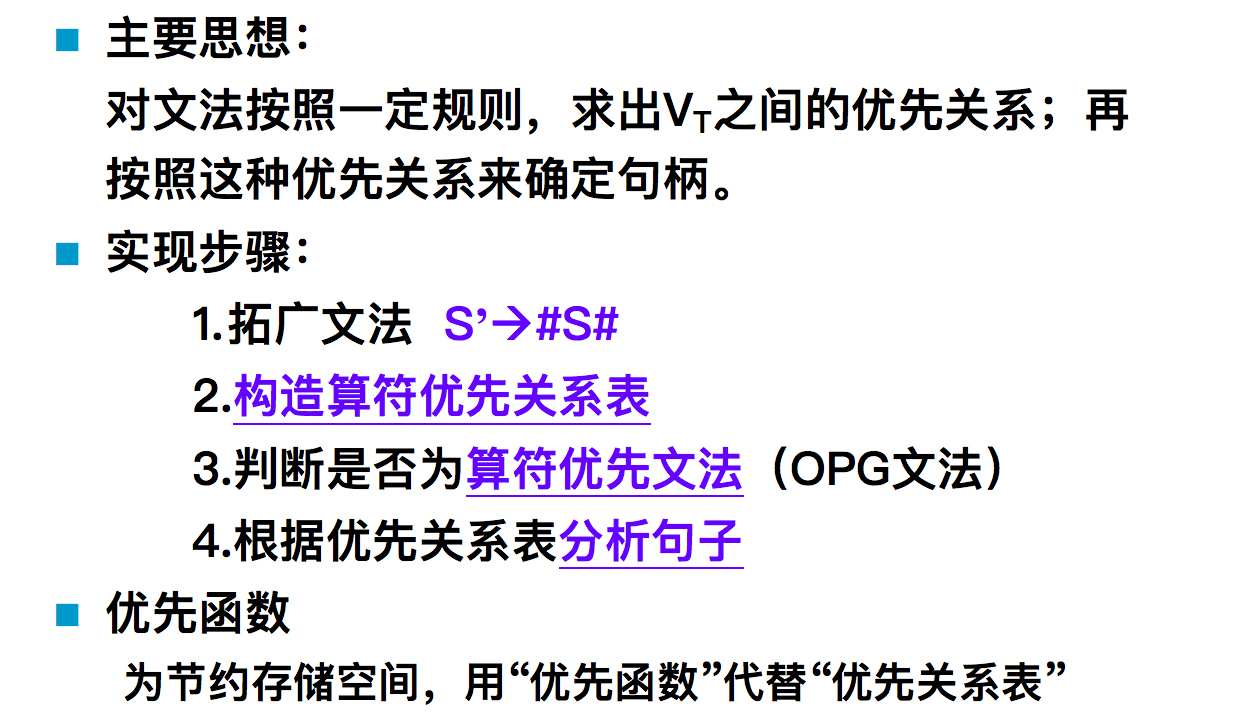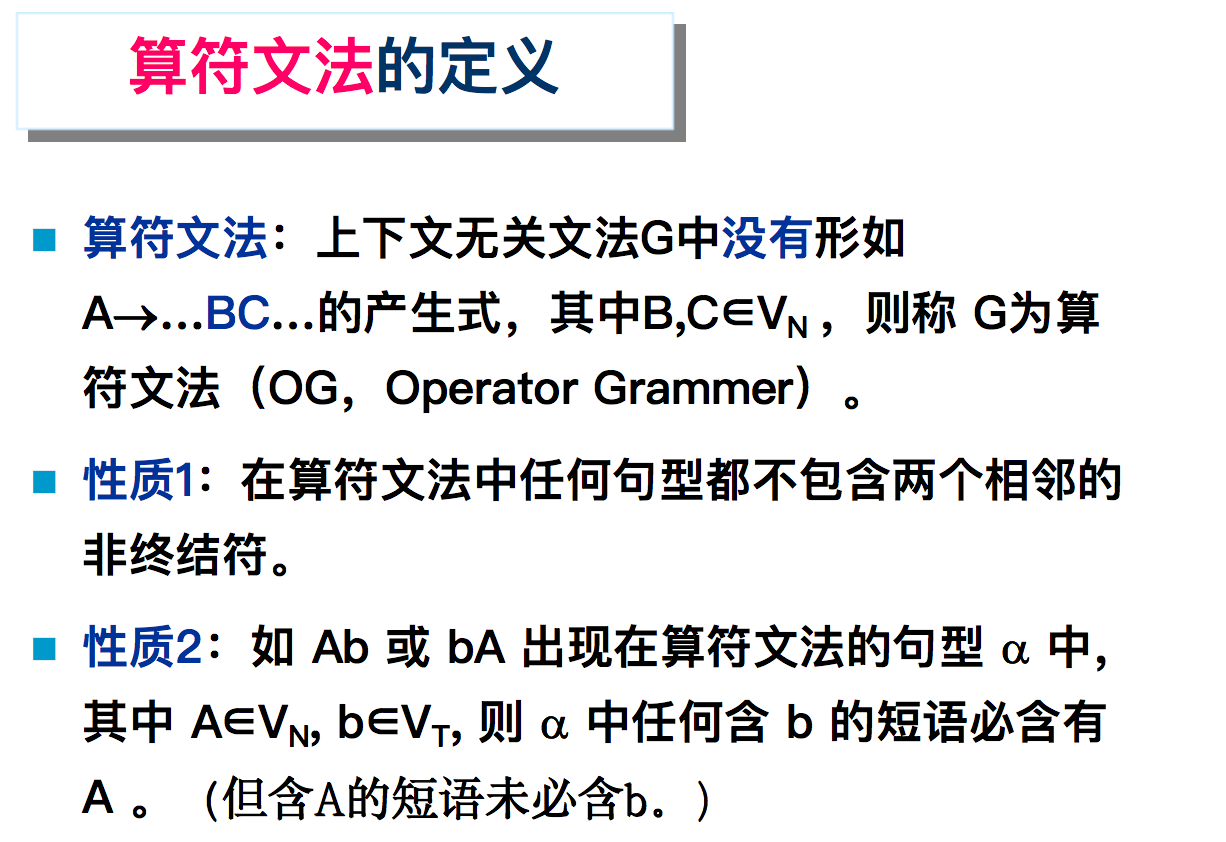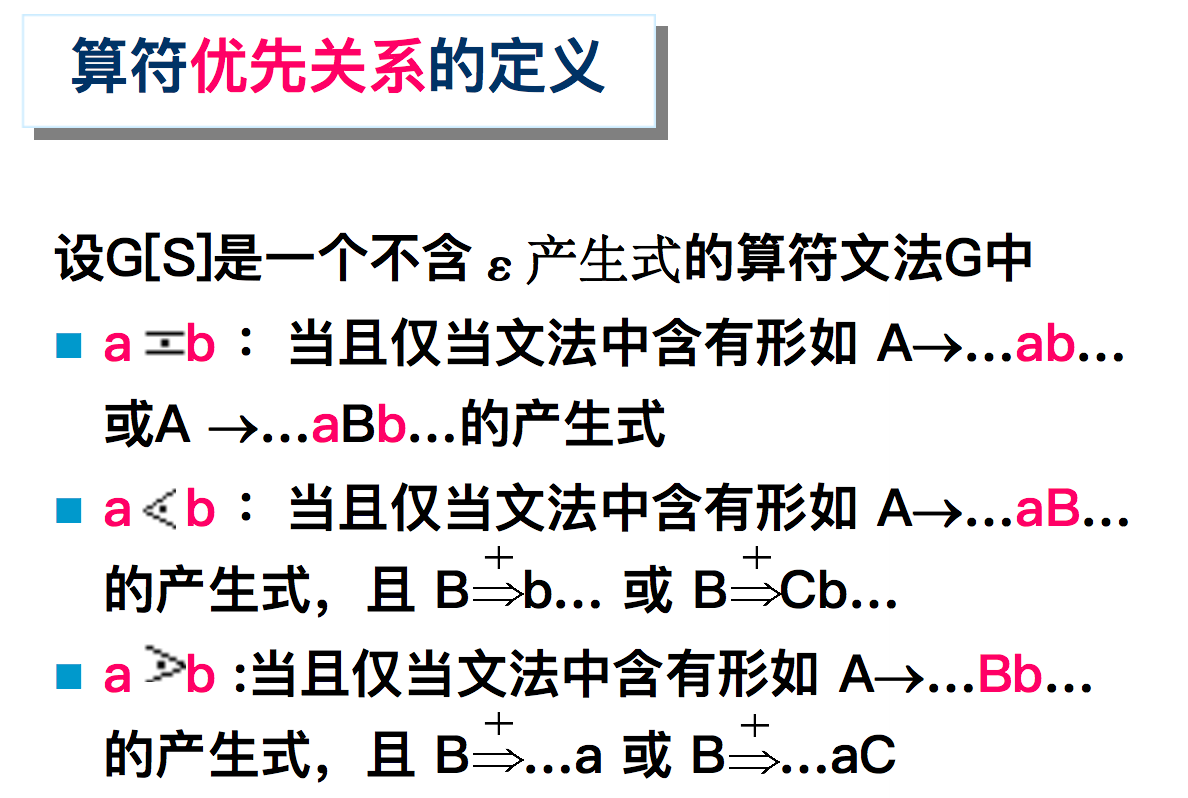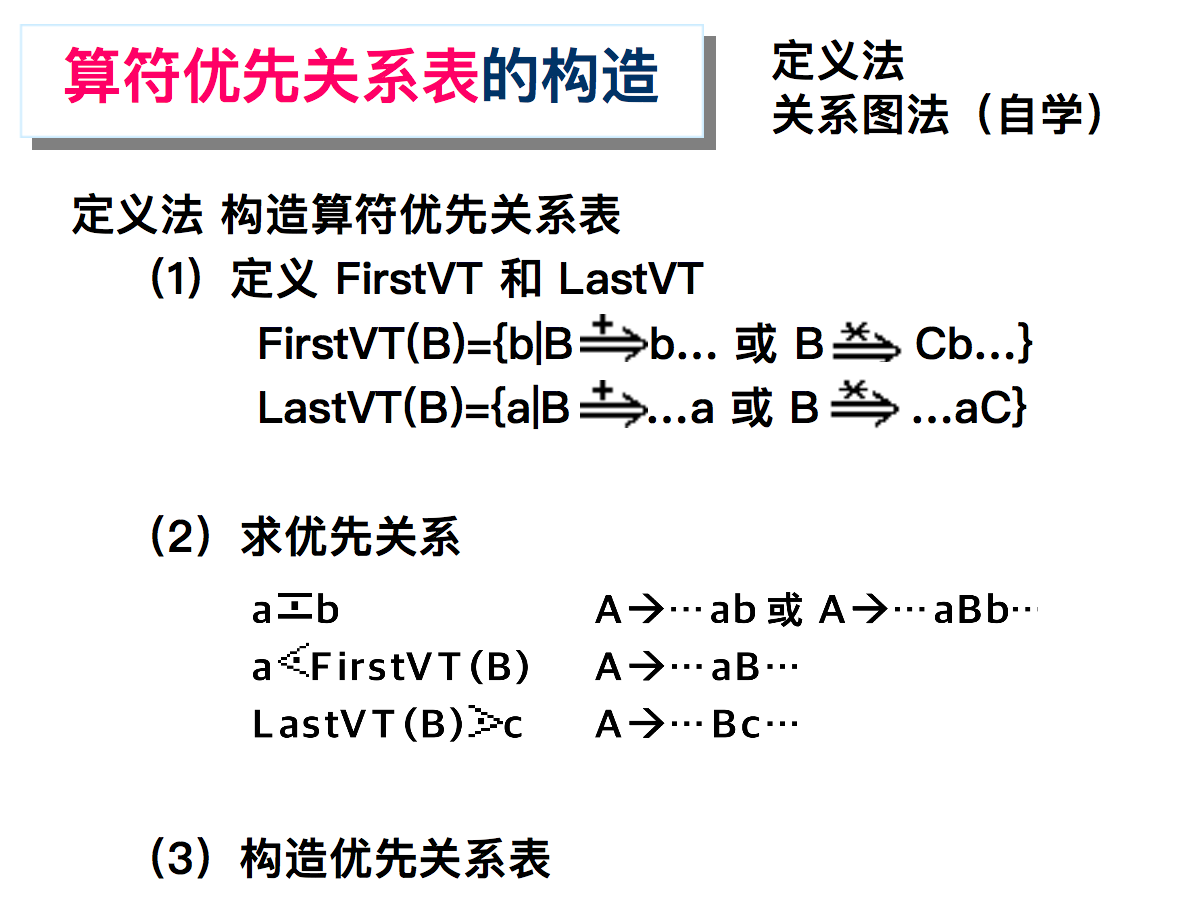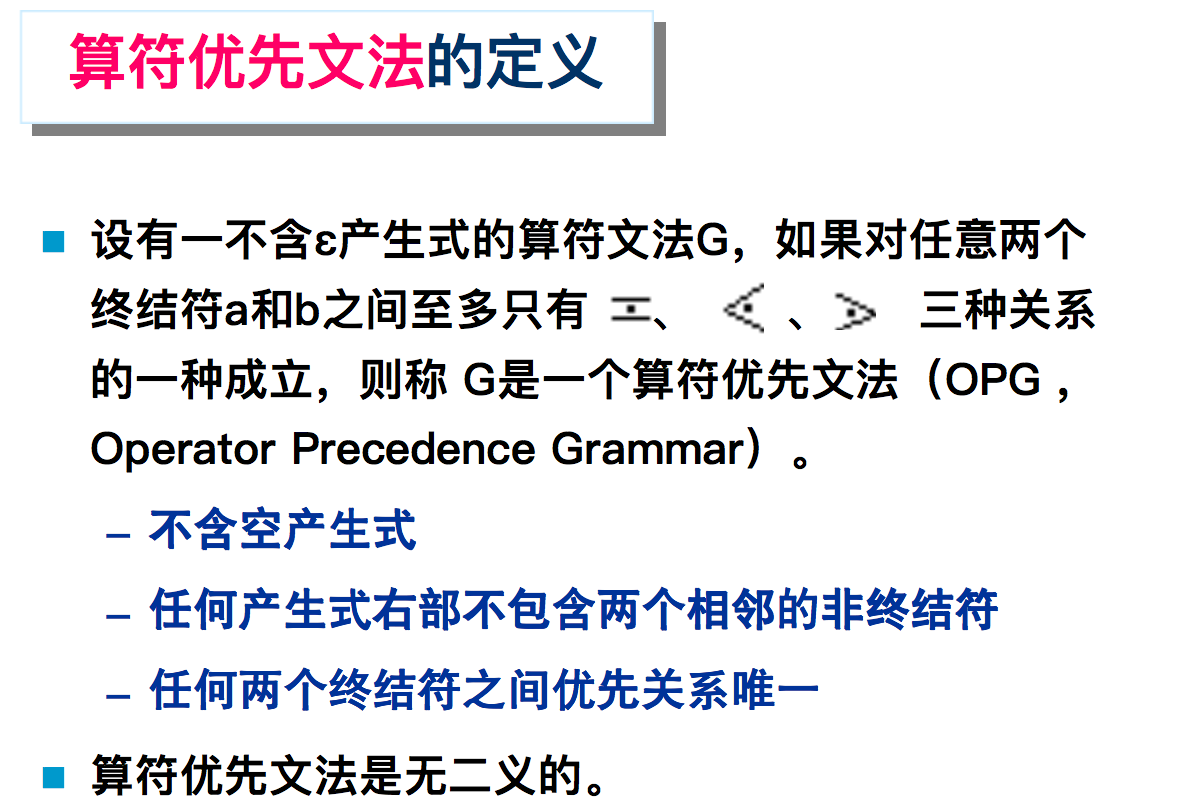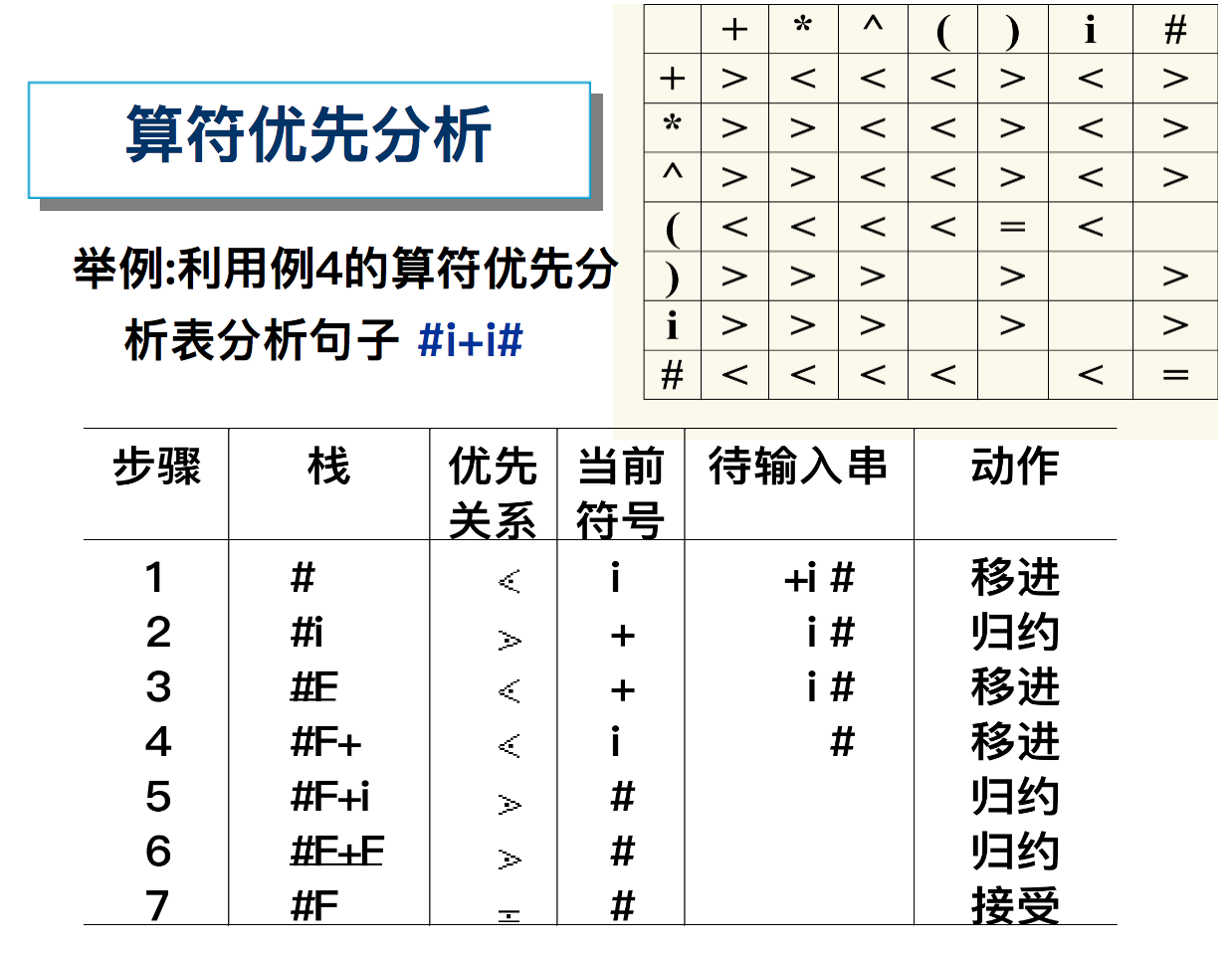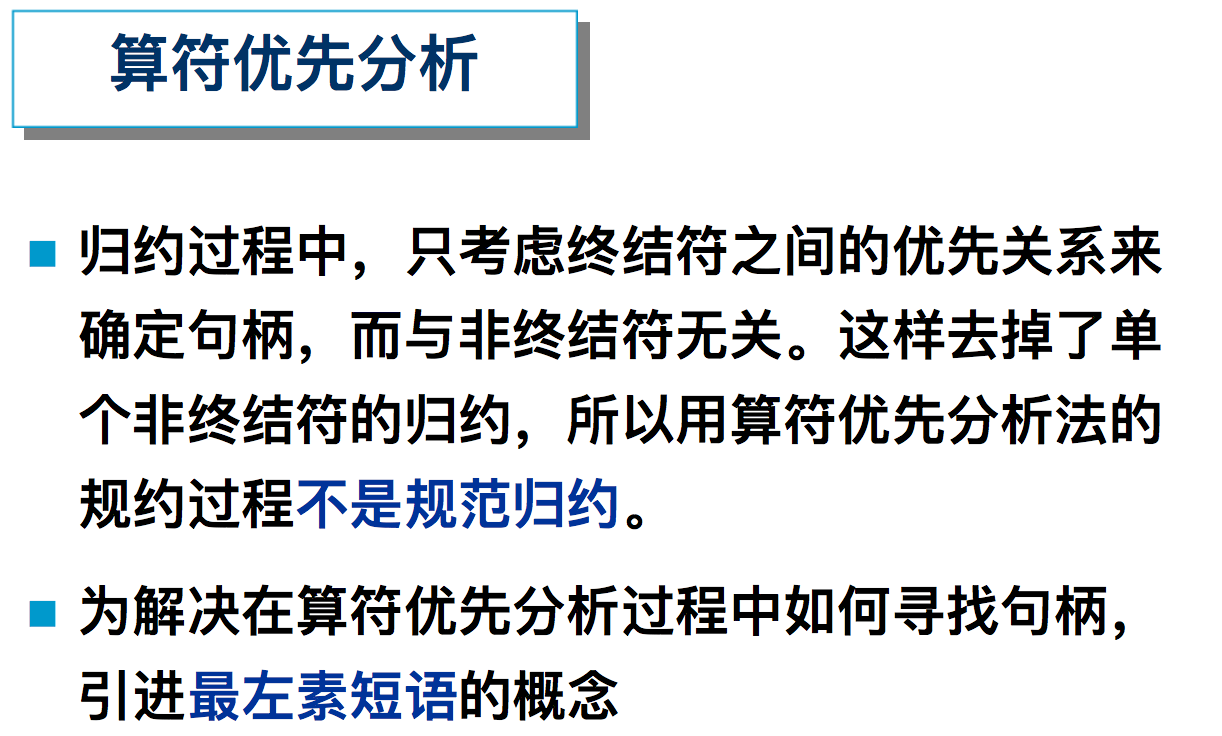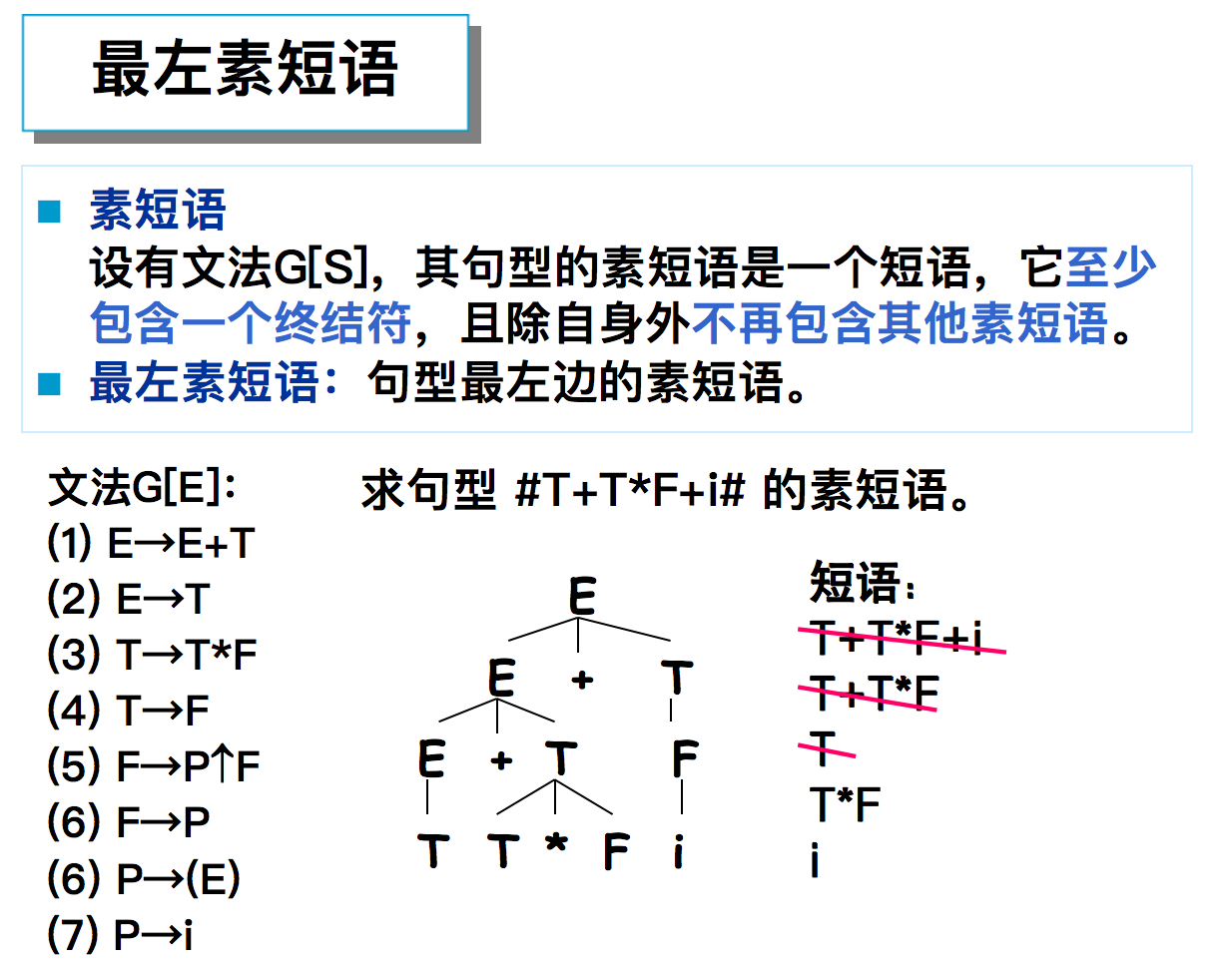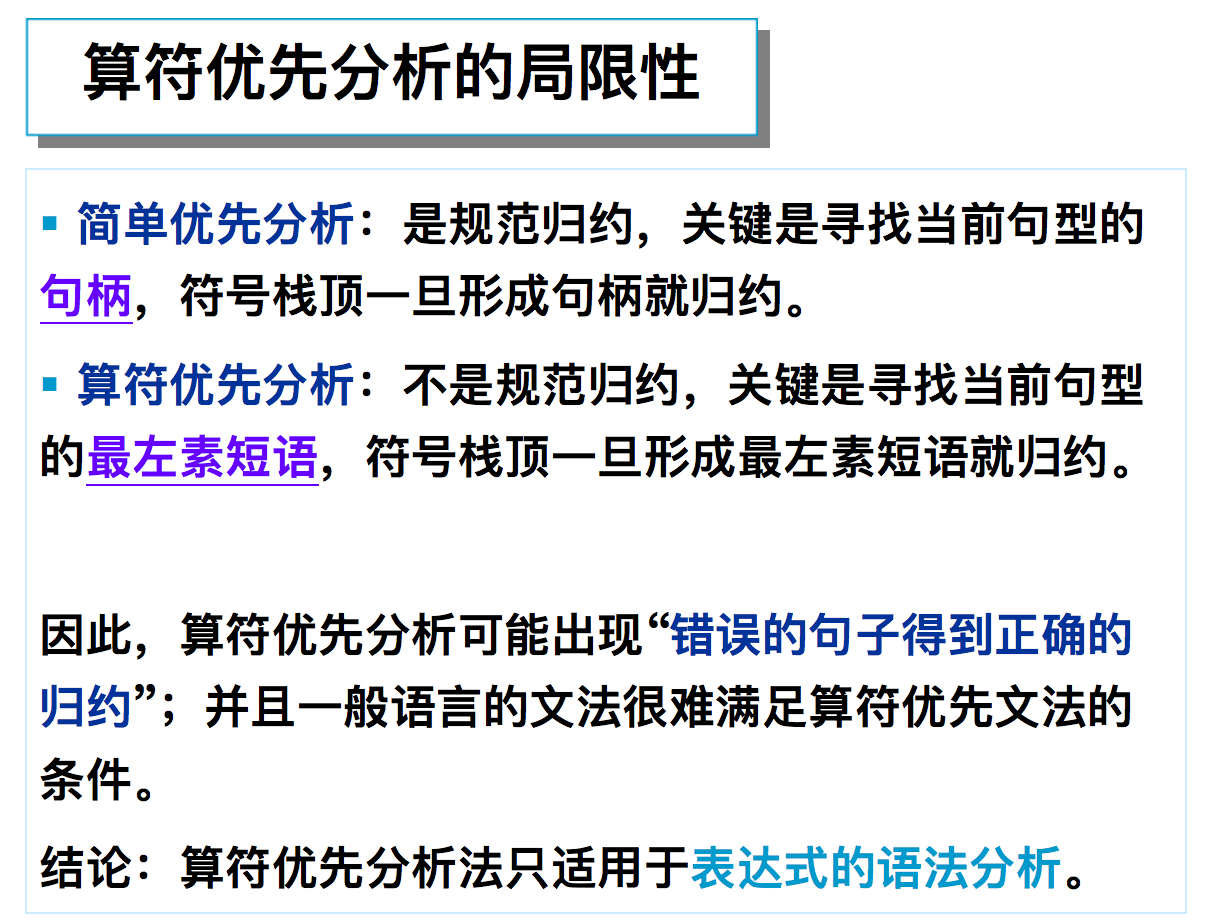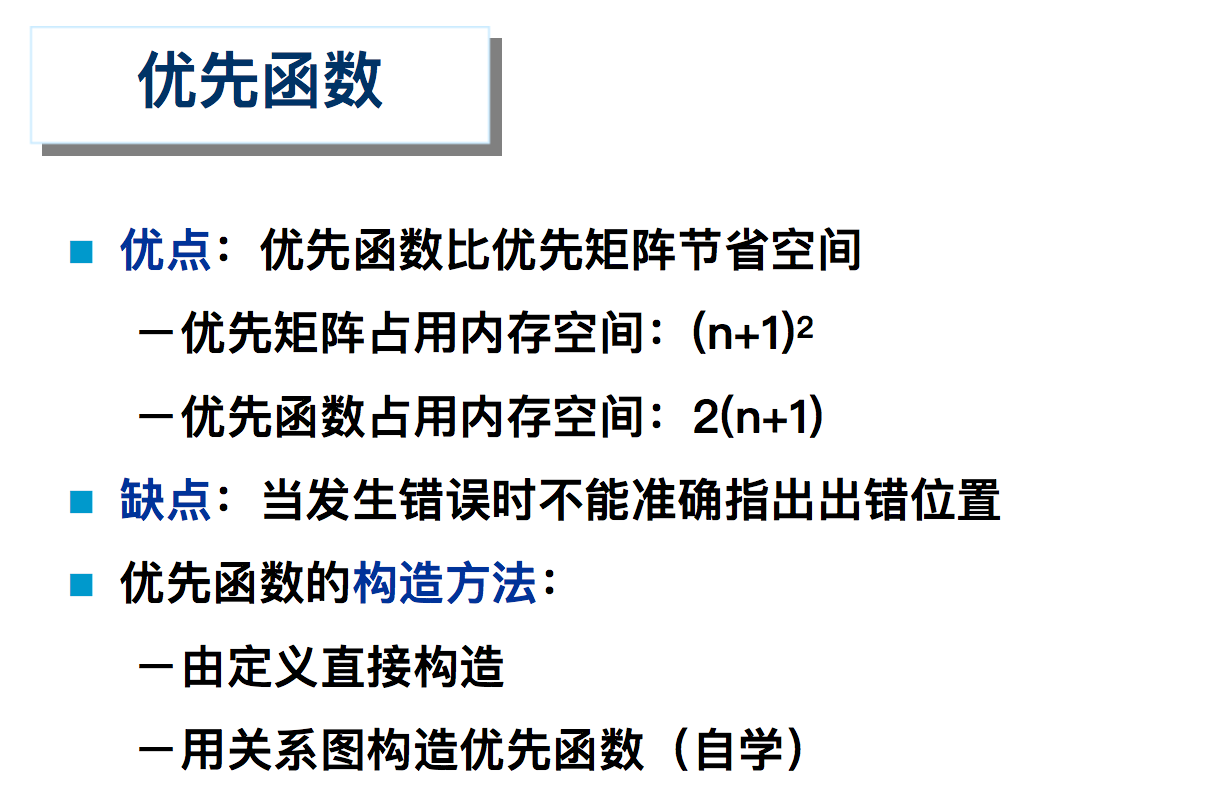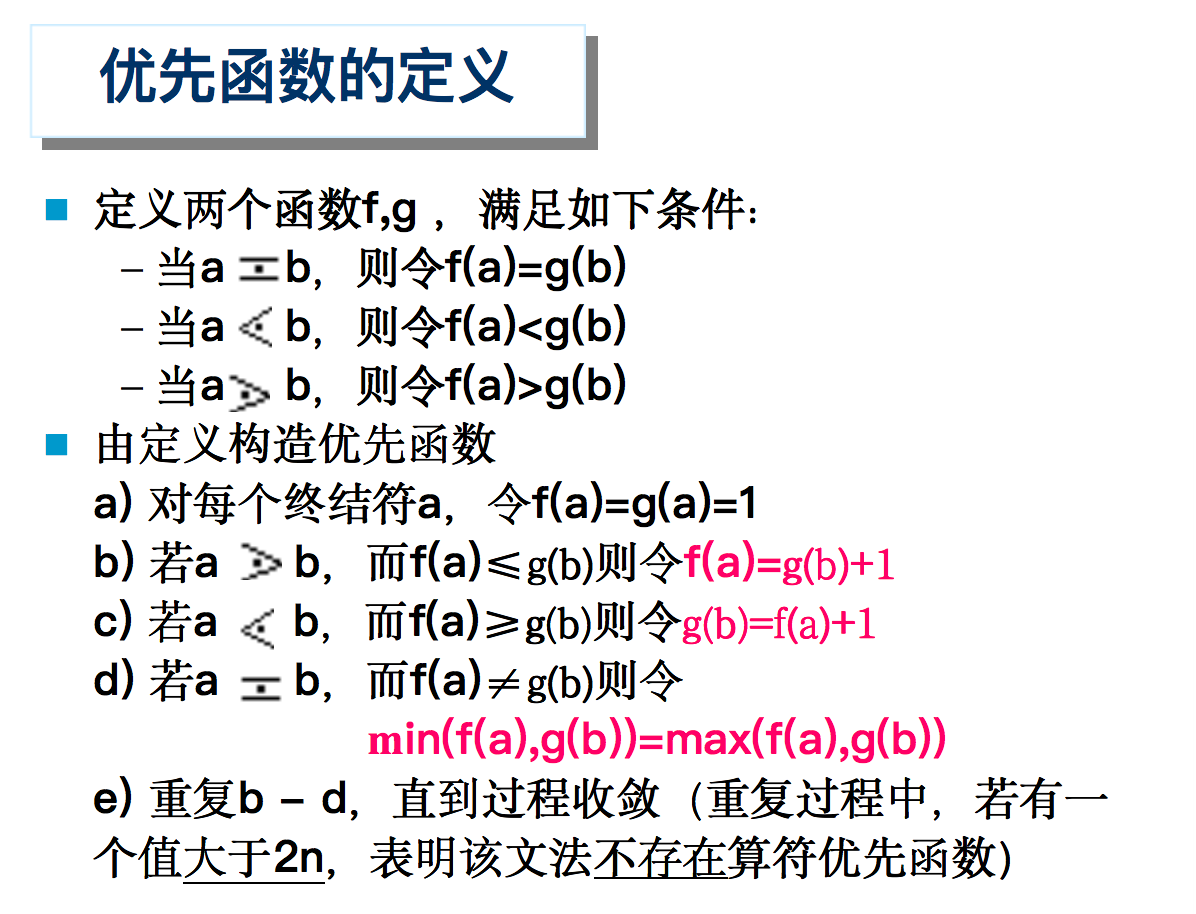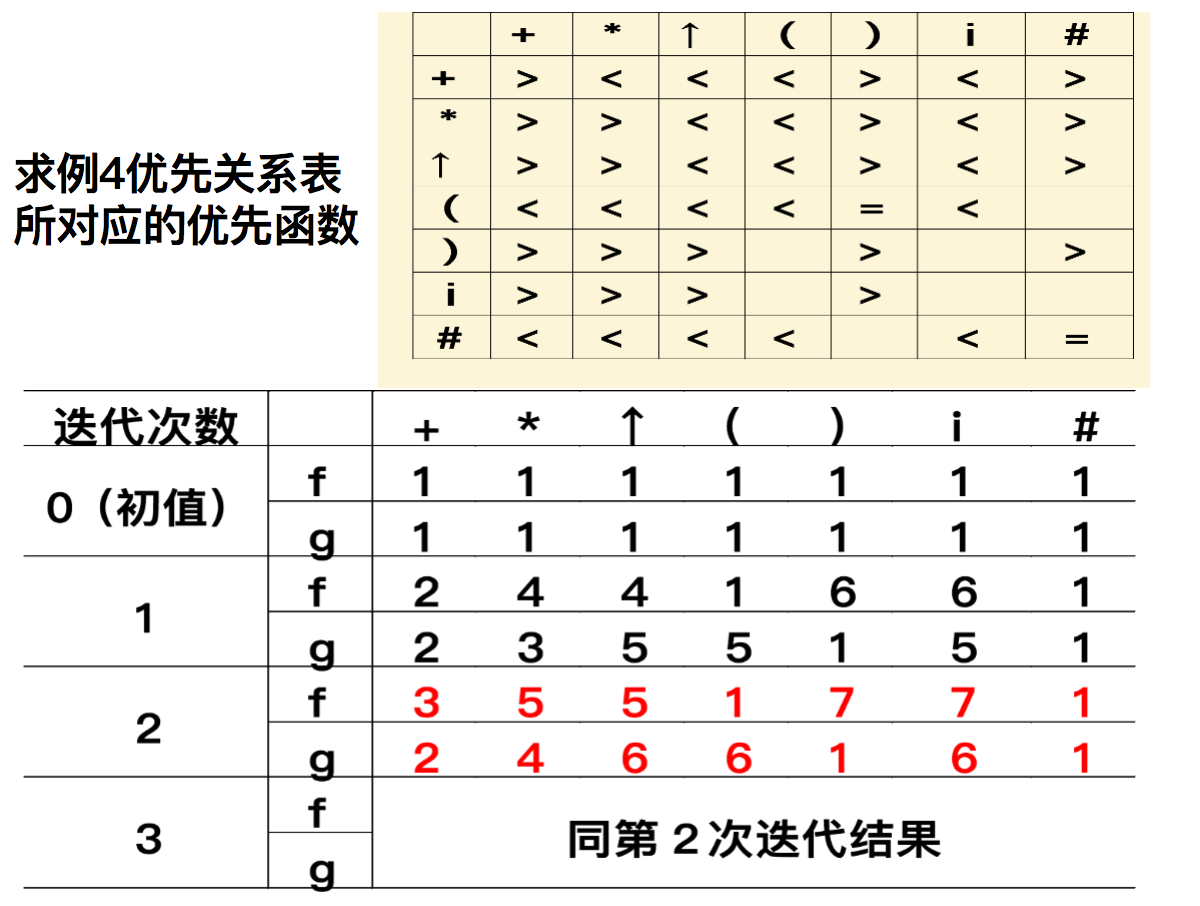• 目录自底向上优先分析概述**简单优先分析法算符优先分析法算符优先分析法概述直观算符优先分析法算符优先文法的定义算符优先关系表的构造算符优先分析算法算符优先分析法的局限LR 分析LR 分析器概述 自底向上优先...

本文参考《编译原理(第三版)》、《龙书》

自底向上优先分析概述

自底向上分析

• 从字符串归约到开始符号 (子孙节点收到根节点)

自底向上分析的关键

• 如何精确定义可归约串并识别 (在规范规约中，可归约串由句柄进行刻画)。对可归约串的不同定义形成不同的自下而上分析方法：
• 规范归约分析中，用句柄来刻画可归约串 (LR 分析, 简单优先分析法)
• 算符优先分析中，是用最左素短语来刻画可归约串

非二义性的文法，它的每个右句型有唯一的句柄

自底向上分析的基本思想 (移进-归约分析)

• 对输入串从左到右扫描，并逐个移入栈中。边移入边分析，一旦栈顶符号串形成某个句型的可归约串，就用该产生式左部的非终极符号代替它，完成进一步归约
• 重复这一过程直到归约到栈中，只剩下开始符号和右边界符’#，则成功。否则报错

( 1 ) S → a A c B e      ( 2 ) A → b      ( 3 ) A → A b      ( 4 ) B → d (1) S\rightarrow aAcBe\ \ \ \ (2) A\rightarrow b\ \ \ \ (3) A\rightarrow Ab\ \ \ \ (4) B\rightarrow d , 对输入串 a b b c d e # abbcde\# 进行自底向上语法分析

• 以按句柄归约为例：自左向右按句柄归约的过程是自顶向下最右推导(规范推导) 的逆过程，这一过程也称为 规范规约 (最左归约)

想象一下语法树；最右推导是从右往左、自顶向下地进行推导；而自底向上分析为从左往右、自底向上地进行分析

• 输入串对应的最右推导为： S ⇒ a A c B e ⇒ a A c d e ⇒ a A b c d e ⇒ a b b c d e S\Rightarrow aAcBe\Rightarrow aAcde\Rightarrow aAbcde\Rightarrow abbcde ，构造它的逆过程，即为规约过程对应的语法树：简单优先分析法

基本思想

• 按一定原则求出所有文法符号 (终结符和非终结符) 之间的优先关系
• 按照文法符号的优先关系确定规约过程中的句柄

简单优先分析法准确、规范，但分析效率较低，实用价值不大

优先关系 定义：

• X = ꞏ Y X=ꞏY ( X X , Y Y 优先性相等) iff 存在产生式规则 A → … X Y … A\rightarrow…XY…
• X < ꞏ Y X<ꞏY ( X X Y Y 优先级小) iff A → … X B … 且 B ⇒ + Y … A\rightarrow…XB…且 B\Rightarrow^+Y…
• X ꞏ > Y Xꞏ>Y ( X X Y Y 优先级大) iff A → … B D … 且 B ⇒ + … X 和 D ⇒ ∗ Y . . . A\rightarrow…BD…且 B\Rightarrow^+…X 和 D\Rightarrow^*Y...

优先关系 与出现的左右次序有关， a < ꞏ b a <ꞏ b 不认为 b ꞏ > a b ꞏ> a

S → b A b , A → ( B ∣ a , B → A a ) \begin{aligned}S&\rightarrow bAb,\\ A&\rightarrow(B | a,\\ B&\rightarrow Aa)\end{aligned}

先求得文法符号之间的优先关系：

• = ꞏ =ꞏ
b = ꞏ A ， A = ꞏ b ， ( = ꞏ B ， A = ꞏ a ， a = ꞏ ) b=ꞏA，A=ꞏb，\\(=ꞏB，\\A=ꞏa，a=ꞏ)
• < ꞏ <ꞏ
• S → b A b S\rightarrow bAb A → ( B ∣ a A\rightarrow (B | a ，得
b < ꞏ ( ， b < ꞏ a b <ꞏ( ，b <ꞏ a
• A → ( B ∣ a A\rightarrow (B | a B → A a ) , A → ( B ∣ a B\rightarrow Aa),A\rightarrow (B | a ，得
( < ꞏ A ， ( < ꞏ ( ， ( < ꞏ a (<ꞏ A， (<ꞏ(，(<ꞏ a
• ꞏ > ꞏ>
• S → b A b S\rightarrow bAb A ⇒ + . . . ) , A ⇒ + . . . B , A ⇒ + a A\Rightarrow^+...),A\Rightarrow^+...B,A\Rightarrow^+a 可得
B ꞏ > b ， a ꞏ > b ， ) ꞏ > b B ꞏ> b ， a ꞏ> b ，) ꞏ> b
• B → A a ) B\rightarrow Aa) A ⇒ + . . . ) , A ⇒ + . . . B , A ⇒ + a A\Rightarrow^+...),A\Rightarrow^+...B,A\Rightarrow^+a 可得
B ꞏ > a , a ꞏ > a , ， ) ꞏ > a B ꞏ> a, a ꞏ> a, ， ) ꞏ> a

上述关系也可由语法树表示：• = ꞏ =ꞏ
( B (B 成为句柄时，它们将同时归约； b A b bAb A a ) Aa) 同理
• < ꞏ <ꞏ
• b ( b( b a ba 出现在某一句型时， ( ( a a 在句柄中而 b b 不在句柄中，因此必须 ( ( a a 先进行归约，所以 b b 的优先级比 ( ( a a
• ( ( (( ( a (a ( A (A 的分析同理
• ꞏ > ꞏ>
• a b ab a a aa 出现在某一句型时，左边的 a a 在句柄中，右边的 a a b b 不可能在句柄中，所以必须左边的 a a 先进行归约；其余关系的分析同理

简单优先关系矩阵• 矩阵中元素要么只有一种关系，要么为空 (该文法的任何句型都不会出现该符号对的相邻关系，在分析中若出现该相邻关系，则出错)
• # \# 优先级小于所有符号(这里指与 # \# 有相邻关系的文法符号)，所有符号的优先级大于 # \#

简单优先文法的定义

• 任意两个文法符号之间最多只有一种优先关系成立
• G G 中任意两个产生式没有相同的右部（归约唯一）

简单优先文法的分析步骤：(栈顶为 a i a_i a i < ꞏ a i + 1 a_i <ꞏa_{i+1} 移进， a i ꞏ > a i + 1 a_i ꞏ>a_{i+1} 归约（已形成句柄）)

• 构造文法的优先关系矩阵，将 G G 规则保存，设置符号栈 S S
• 将输入串 a 1 a 2 … a n # a_1a_2…a_n\# 逐个移入符号栈 S S 中，直到栈顶符号 a i ꞏ > a i + 1 a_i ꞏ>a_{i+1} 为止。
• 栈顶 a i a_i 为句柄尾，由此向左在栈中找句柄开头符号 a k a_k （ 找到 a k − 1 < ꞏ a k a_{k-1} <ꞏa_k 为止）
• 由句柄 a k a k + 1 … a i a_ka_{k+1}…a_i 在产生式中查找右部为 a k … a i a_k…a_i 的产生式，若找到则用相应左部代替句柄（归约），否则出错，串不是合法句子

算符优先分析法

算符优先分析法概述

• 自底向上分析的核心就是寻找可归约串(短语)，而算符优先分析法是依据四则运算过程而设计的一种语言分析方法，它将一类特殊的短语作为可归约串(最左素短语)
• 只考虑算符 (终结符) 之间的优先关系 (不考虑 V N V_N 的优先关系)

算符优先分析法虽然不是规范规约，但分析速度快 (仅适用于表达式语法的分析)

G [ E ] : E → E + E ∣ E ∗ E ∣ ( E ) ∣ i d （ 歧 义 文 法 ） G[E]:E \rightarrow E+E | E*E |(E)|id（歧义文法）

• 句子 i d + i d ∗ i d id+id*id 有两个不同的规范归约，即归约中句柄不唯一• 产生歧义的原因：没有定义 + + ∗ * 的优先关系
• 因此，算符优先分析法的关键即为：如何定义任意两个可能相邻出现的终极符 a a b b 之间的优先关系

直观算符优先分析法

在算术表达式求值过程中，运算次序只与运算符有关，而与运算对象无关 (先乘除后加减)，因此直观算符优先分析法的关键是：

• 对文法 G G 人为规定其算符优先顺序，即给出优先级别和同一级别中的结合性质 (左结合、右结合)
• = ꞏ =ꞏ
• < ꞏ <ꞏ
• ꞏ > ꞏ>

优先关系 与出现的左右次序有关，例如，通常表达式中运算符的优先关系有 + ꞏ > − +ꞏ>- ，但没有 − < ꞏ + -<ꞏ+ ；有 ( = ꞏ ) (=ꞏ) ，但没有 ) ꞏ = ( )ꞏ=(

给出一个表达式的二义性文法：
E → E + E ∣ E − E ∣ E ∗ E ∣ E / E ∣ E ↑ E ∣ ( E ) ∣ i E\rightarrow E+E|E-E|E*E|E/E|E\uparrow E|(E)|i

运算对象的终结符 i i 优先级最高，其他运算符按计算顺序规定如下优先级和结合性：

• ↑ \uparrow 优先级最高，遵循右结合 (归约时从右往左归约)，即 i 1 ↑ i 2 ↑ i 3 i_1\uparrow i_2\uparrow i_3 i 2 ↑ i 3 i_2\uparrow i_3 先归约
↑ < ꞏ ↑ \uparrow<ꞏ\uparrow

• ∗ * / / 的优先级低于 ↑ \uparrow ，服从左结合：
∗ ꞏ > ∗ , ∗ ꞏ > / , / ꞏ > / , / ꞏ > ∗ *ꞏ>*,*ꞏ>/,/ꞏ>/,/ꞏ>*

• + + − - 的优先级最低，服从左结合：
+ ꞏ > + , + ꞏ > − , − ꞏ > − , − ꞏ > + +ꞏ>+,+ꞏ>-,-ꞏ>-,-ꞏ>+

• ( ( ) ) 规定，括号的优先级大于括号外的运算符，小于括号内的运算符。内括号的优先级大于外括号

• # \# 规定，与它相邻的任何运算符的优先性都比它大

• 综上所述，可以得到如下的算符优先关系表：有了算符优先关系表， i 1 + i 2 ∗ i 3 i_1+i_2*i_3 的归约过程就是唯一确定的了。即当使用移进-归约分析法时，栈中出现 # E + E \#E+E ，可归约为 # E \#E ，但当输入符为 ∗ * ，而 + < ⋅ ∗ +<\cdot* ，这时句柄尾还未找到，所以应该移进而非归约

算符优先文法的定义

算符优先分析法只适用于算符优先文法

算符文法 （Operater Grammar） ：

• 设有文法 G G ，若 G G 中没有形如 A → … B C … A\rightarrow…BC… 的产生式，其中 B , C ∈ V N B, C∈V_N ，则称 G G 为算符文法，也称为 O G OG 文法

算符文法具有两个重要性质

• O G OG 文法中，任何句型都不包含两个相邻的非终结符• A b Ab b A bA 出现在算符文法的句型 β β 中， A ∈ V N ， b ∈ V T A ∈V_N，b ∈V_T ，则 β β 中任何含 b b 的短语必含有 A A任意两个终结符号之间的优先关系

G G 是一个不含 ε ε 规则的 O G OG 文法 a 、 b a、b 是任意两个终结符， A 、 B 、 C A、B、C 是非终结符，算符优先关系 = ꞏ , < ꞏ , ꞏ > =ꞏ, <ꞏ, ꞏ> 定义如下：

• a = ꞏ b a =ꞏb 当且仅当 G G 中含有形如 A → … a b … A\rightarrow…ab… A → … a B b … A\rightarrow…aBb… 规则
• a < ꞏ b a <ꞏb 当且仅当 G G 中含有形如 A → … a B … A\rightarrow…aB… 产生式且
B ⇒ + b … B\Rightarrow^+ b… B ⇒ + C b … B\Rightarrow^+ Cb…
• a ꞏ > b a ꞏ> b 当且仅当 G G 中含有形如 A → … B b … A\rightarrow…Bb… 产生式且
B ⇒ + … a B\Rightarrow^+ …a B ⇒ + … a C B\Rightarrow^+ …aC

对应的语法树• ( a a ) δ \delta ε \varepsilon C C a , b a,b 在同一可归约串中，同时归约，因此优先级相同
• ( b b ) a , b a, b 不在同一可归约串中， b b 先归约，所以 a < ꞏ b a<ꞏb
• ( c c ) a , b a, b 不在同一可归约串中， a a 先归约，所以 a ꞏ > b aꞏ>b

算符优先文法 (Operater Precedence)

• 设有一个不含 ε ε 规则的算符文法 G G ，如果任意两个终结符对 ( a , b ) (a, b) < ꞏ , ꞏ > , = ꞏ <ꞏ, ꞏ>, =ꞏ 三种关系中只有一种成立，则称 G G 是算符优先文法，也称 O P G OPG 文法
• 也就是说，如果 a N b aNb a b ab 出现在句型 r r 中，则
• a < ꞏ b a<ꞏb ，则在 r r 中必含有 b b 而不含 a a 的短语存在
• a ꞏ > b aꞏ>b ，则在 r r 中必含有 a a 而不含 b b 的短语存在
• a = ꞏ b a=ꞏb ，则在 r r 中含有 a a 的短语必含有 b b

判断： G [ E ] G[E] 是否是OG，是否是OPG文法
E → E + E ∣ E ∗ E ∣ ( E ) ∣ i d E \rightarrow E+E | E*E | (E) | id

• 是 OG，不是 OPG• 而： G ′ [ E ] ： E → E + T ∣ T , T → T ∗ F ∣ F , F → ( E ) ∣ i d G'[E] ：E \rightarrow E+T | T,T \rightarrow T*F | F,F \rightarrow (E) | id 是 OPG 文法

算符优先关系表的构造

G G 是一个不含 ε ε 规则的 O G OG 文法 a 、 b a、b 是任意两个终结符， A 、 B 、 C A、B、C 是非终结符，算符优先关系 = ꞏ , < ꞏ , ꞏ > =ꞏ, <ꞏ, ꞏ> 定义如下：

• a = ꞏ b a =ꞏb 当且仅当 G G 中含有形如 A → … a b … A\rightarrow…ab… A → … a B b … A\rightarrow…aBb… 规则
• a < ꞏ b a <ꞏb 当且仅当 G G 中含有形如 A → … a B … A\rightarrow…aB… 产生式且
B ⇒ + b … B\Rightarrow^+ b… B ⇒ + C b … B\Rightarrow^+ Cb…
• a ꞏ > b a ꞏ> b 当且仅当 G G 中含有形如 A → … B b … A\rightarrow…Bb… 产生式且
B ⇒ + … a B\Rightarrow^+ …a B ⇒ + … a C B\Rightarrow^+ …aC

根据优先关系定义，可按如下方法直接构造优先关系表

• 首先，对 G G 中每个非终结符 A A ，定义两个集合 (最左终结符号集、最右终结符号集)：
F I R S T V T ( A ) = { b ∣ A ⇒ + b … 或 A ⇒ + B b … , b ∈ V T , B ∈ V N } L A S T V T ( A ) = { a ∣ A ⇒ + … a 或 A ⇒ + … a B , a ∈ V T , B ∈ V N } FIRSTVT(A)=\{b| A \Rightarrow^+ b…或A \Rightarrow^+ Bb…, b∈V_T, B∈V_N\}\\ LASTVT(A)=\{a | A \Rightarrow^+ …a或 A\Rightarrow^+ …aB, a∈V_T, B∈V_N\}
• 4 种优先关系：
• 对形如 A → … a b … , A → … a B b … , A\rightarrow…ab…, A\rightarrow…aBb…, a = ꞏ b a =ꞏ b
• 对形如 A → … a B … , A\rightarrow…aB…, a < ꞏ b , b ∈ F I R S T V T ( B ) a <ꞏb, b∈FIRSTVT(B)
• 对形如 A → … B b … , A\rightarrow…Bb…, a ꞏ > b , a ∈ L A S T V T ( B ) a ꞏ> b, a∈LASTVT(B)
• 对文法开始符号 S S ，有 # < ꞏ F I R S T V T ( S ) , L A S T V T ( S ) ꞏ > # \# <ꞏ FIRSTVT(S), LASTVT(S) ꞏ> \# ，且对 # S # \#S\# ，有 # = ꞏ # \# =ꞏ \#

求得每个 V N V_N F I R S T V T ( A ) FIRSTVT(A)

• (1) 若有产生式 A → a … A\rightarrow a… A → B a … A\rightarrow Ba… , 则 a ∈ F I R S T V T ( A ) a∈FIRSTVT(A)
• (2) 若 a ∈ F I R S T V T ( B ) a∈FIRSTVT(B) ，且有产生式 A → B … A\rightarrow B… ，则 a ∈ F I R S T V T ( A ) a∈FIRSTVT(A)

基于上述两条规则，可以设计如下算法

• 先建立一个布尔数组 F [ m , n ] F[m,n] ( m m 为非终结符个数， n n 为终结符个数)。算法的目的是使 ∀ a , A ,      a ∈ F I R S T V T ( A )   当 且 仅 当   F [ A , a ] 为 真 \forall a,A,\ \ \ \ a\in FIRSTVT(A)\ 当且仅当\ F[A,a]为真
• 首先按规则 (1) 对每个数组元素赋初值。遍历这些初值，若 F [ A , a ] F[A,a] 为真，将 ( A , a ) (A,a) 入栈
• 然后栈顶元素出栈，设为 ( B , a ) (B,a) ，再用规则 (2) 检查所有产生式，若有形如 A → B … A\rightarrow B… 的产生式，而 F [ A , a ] F[A,a] 为假，则将其置为真， ( A , a ) (A,a) 入栈… 重复此步骤直至栈空求得每个 V N V_N L A S T V T ( A ) LASTVT(A)

• 若有产生式 A → … a A\rightarrow …a A → … a B A\rightarrow …aB , 则 a ∈ L A S T V T ( A ) a∈LASTVT(A)
• a ∈ L A S T V T ( B ) a∈LASTVT(B) ，且有产生式 A → … B A\rightarrow …B ，则 a ∈ L A S T V T ( A ) a∈LASTVT(A)

E ′ → # E # E → E + T ∣ T T → T ∗ F ∣ F F → P ↑ F ∣ P P → ( E ) P → i \begin{aligned}E'&\rightarrow \# E \#\\ E &\rightarrow E+T | T \\ T &\rightarrow T*F | F \\ F &\rightarrow P ↑ F | P \\ P &\rightarrow (E)\\ P &\rightarrow i\end{aligned} 求所有 V N V_N F I R S T V T FIRSTVT

• 第一次扫描后，STACK 栈的初值为：• F → P ， T → F , E → T F\rightarrow P，T \rightarrow F, E \rightarrow T ，栈顶 (6) 的内容逐次改变为: ( F , i ) , ( T , i ) , ( E , i ) (F, i), (T, i), (E, i) ，之后栈中只剩下5个元素，之后的具体过程同理，过程如下图所示：• 最终得到结果：也可由简单关系图形求得：构造 G G 的优先关系表算法

1. 为每一个 A ∈ V N A∈V_N ，计算 F I R S T V T ( A ) FIRSTVT(A) L A S T V T ( A ) LASTVT(A)
2. 执行程序
for(每个产生式 A -> x1x2…xn)
{
for(i = 1; i <= n - 1; i++)
{
if(xi∈VT && xi+1∈VT)
置 xi ꞏ= xi+1
if(i <= n-2 && xi∈VT && xi+1∈VN && xi+2∈VT)
置 xi ꞏ= xi+2
if(xi∈VT, xi+1∈VN)
{
for(FIRSTVT(xi+1)中的每个变量b)
置 xi ꞏ< b
}
if(xi∈VN, xi+1∈VT)
{
for(LASTVT(xi)中的每个变量a)
置 a >ꞏ xi
}
}
}

1. F I R S T V T ( S ) FIRSTVT(S) 中的所有 b b ，置 # ꞏ < b \#ꞏ<b ；对 L A S T V T ( S ) LASTVT(S) 中的所有 a a ，置 a ꞏ > # aꞏ>\# ; 置 # ꞏ = # \#ꞏ=\#

G [ E ] : E → E + T ∣ T , T → T ∗ F ∣ F , F → ( E ) ∣ i d ， \begin{aligned}G[E]: E&\rightarrow E+T | T, \\ T&\rightarrow T*F | F, \\ F&\rightarrow (E) | id，\end{aligned} 构造出算符优先关系表算符优先关系表+ ⋅ > + +\cdot>+ ，因此 + + 满足左结合

算符优先分析算法

• 算符优先分析算法不是一种规范归约。即：不是用句柄，而是用最左素短语刻画可归约串

最左素短语

• 句型的素短语：至少包含一个 V T V_T 的短语，并且除自身之外不再包含其它的素短语
• 最左边的素短语称为最左素短语

算符优先分析算法是按照终结符的优先性进行比较的，因此需要定义至少含有一个终结符的短语，以便进行算符比较，确定可归约串。而算符优先分析算法又是从左向右扫描的，因此需要定义最左素短语来确定下一步需要归约的对象

G [ E ] : E → E + T ∣ T , T → T ∗ F ∣ F , F → ( E ) ∣ i d ， \begin{aligned}G[E]: E&\rightarrow E+T | T, \\ T&\rightarrow T*F | F, \\ F&\rightarrow (E) | id，\end{aligned} 考虑 G [ E ] G[E] 的句型： T + T ∗ F + i d T+T*F+id短语对应 V N V_N
T + T ∗ F + i d T+T*F+id E E
T + T ∗ F T+T*F E E
T T E E
T ∗ F T*F T T
i d id P , F , T P,F,T
• 素短语： T ∗ F T*F , i d id
• 最左素短语： T ∗ F T*F T T 是该句型的句柄，但不是最左素短语）

注意，语法分析的输出结果就是语法树，因此实际操作中不可能有一棵语法树来帮助寻找最左素短语，所以我们需要另一种识别最左素短语的方法

识别最左素短语的方法

• 算符文法句型的一般形式为：
# N 1 a 1 N 2 a 2 … N n a n N n + 1 # \#N_1a_1N_2a_2…N_na_nN_{n+1}\# 其中 N i ( 1 ≤ i ≤ n + 1 ) N_i (1\leq i\leq n+1) 为非终结符或空， a i ∈ V T ( 1 ≤ i ≤ n ) a_i∈V_T(1\leq i\leq n)
• 最左素短语定理：一个算符优先文法 G G 的任何句型的最左素短语是满足下列条件的最左子串 N i a i N i + 1 a i + 1 … N j a j N j + 1 N_ia_iN_{i+1}a_{i+1}…N_ja_jN_{j+1}
• a i − 1 < ꞏ a i a_{i-1} <ꞏa_i
• a i = ꞏ a i + 1 = ꞏ … = ꞏ a j a_i =ꞏ a_{i+1} =ꞏ …=ꞏ a_j
• a j ꞏ > a j + 1 a_j ꞏ> a_{j+1}

(注意： O G OG 的任何句型中 V T V_T V N V_N 相邻时，含 V T V_T 的短语必含相邻的 V N V_N (性质2)。因此，出现在 a i a_i 左端的 N i N_i 和出现在 a j a_j 右端的 N j + 1 N_{j+1} 是属于素短语的；也就是说，只要找到终结符形成的可归约串，那么完全不管同时归约了哪些非终结符)

i 1 ∗ ( i 2 + i 3 ) # # < ꞏ i 1 ꞏ > ∗ < ꞏ ( < ꞏ i 2 ꞏ > + < ꞏ i 3 ꞏ > ) ꞏ > # i_1 *( i_2 + i_3) \#\\ \#<ꞏi_1 ꞏ>*<ꞏ(<ꞏ i_2 ꞏ>+<ꞏ i_3ꞏ>) ꞏ>\#

因此， i 1 ， i 2 ， i 3 i_1，i_2，i_3 是素短语； i 1 i_1 是最左素短语

算符优先分析算法

• 在分析过程中设置一个符号栈 S S ，存放归约或待形成最左素短语的符号串， a a 存放当前读入符号
• 采用移进-归约法，如果栈顶的一部分子串为最左素短语，则只需将它与规则右部比较，自左至右，终结符对终结符，非终结符对非终结符，只要对应位置上的终结符相同，同一位置上都有非终结符，就进行归约
• 算法工作完毕时 (读到句子结束符 # \# )，栈中只剩下 # N # \#N\# (即只剩一个非终结符 (不一定是文法开始符号 S S ))
k = 1;	// 栈顶指针
S[k] = '#';
do{
if(S[k]∈VT)
j = k;
else	// 算符优先文法不允许连续出现非终结符，因此 j 指向最靠近栈顶的终结符
j = k - 1;

// 如果栈顶的终结符优先级大于栈外面的输入字符a，则从栈顶开始向栈底扫描，找到最左素短语的头
while(S[j]> a)
{
do{
Q = S[j];
if(S[j - 1]∈VT)
j--;
else
j = j - 2;
}while(s[j] <ꞏ Q || s[j] =ꞏ Q);
把 S[j+1]...S[k] 归约为某个 N;	// 归约最左素短语
k = j + 1;
s[k] = N;
}

// 如果栈顶的终结符优先级小于等于栈外面的输入字符a，则需要将a入栈
if(s[j] <ꞏ a || s[j] =ꞏ a)
{
k++;
s[k] = a;
}else
ERROR;	// 两个终结符之间没有优先关系，报错
}while(a != '#');	// 读入 #，所有单词处理完成• 由上面的例子也可以清晰地看出，算符优先分析算法只考虑终结符之间的优先关系来确定句柄，而与非终结符无关
• 上例中的分析树和语法树的区别在于缺少了单非终结符的归约 (回想一下，在算符优先分析算法中使用最左素短语进行归约，而最左素短语必含有终结符，因此不可能对单个非终结符进行归约)
• 因此，算符优先分析算法得到的不是语法树，只是语法树的框架

算符优先分析法的局限

• 由于算符优先分析法跳过了所有非终结符之间的归约，算符优先分析法比规范归约快得多。这既是它的优点，也是缺点
• 缺点：算符优先分析法在进行归约时，只要求从左到右的终结符与产生式右部的终结符相同，忽略 V N V_N 在归约过程中的作用，存在某种危险性，可能把错误的输入串误认为是句子

LR 分析

LR 分析方法是当前最广义的无回溯的 “移进-归约” 方法，根据历史、现实、展望三者信息来确定栈顶符号串是否形成句柄

• LR分析法 分析速度快；报错准确；适用的范围大，对文法的要求低，无须消除左递归，也无须消除左公共因子。除二义性文法外，绝大多数上下文无关文法描述的程序设计语言都可用 LR 语法分析器予以识别
• 目前大多数编译程序的语法分析器都采用这种分析方法
• 主要缺点是对于一个使用语言文法的分析器构造工作量很大；对于 LR( k k ) 分析来说， k k 越大，构造越复杂。很多实用的编译程序在采用 LR 分析器时都是借助 yacc (Yet Another Compiler Compiler). yacc 能够通过给定语法，自动构造出分析器

L R ( k ) LR(k) 分析

• L L 是指从左至右扫描输入符号串
• R R 是指构造一个最右推导的逆过程（规范归约 Reduce）
• k k 是指为了作出分析决定而向前看的输入符号的个数
• 只须根据 分析栈已移进和归约出的全部文法符号至多向右查看输入串的 k k ( k ≥ 0 k\geq 0 ) 个输入符号，就能唯一确定相当于某一产生式右部符号的句柄是否已在分析栈的顶部形成，从而确定分析器的动作是移进还是归约，以及用哪个产生式进行归约 (查 LR 分析表)• 当前扫描到 X n + 1 X_{n+1} ，向前查看 k k 个符号，(通过查 LR 分析表) 来确定是把 X n + 1 X_{n+1} 移进栈，还是把 X i … X n X_i…X_n 作为句柄进行归约

LR 分析器概述

LR 分析的结构

• LR 分析表 (由两个矩阵组成) ：依赖于具体文法，例如，如果文法为 L R ( 0 ) LR(0) 文法，就要构造 L R ( 0 ) LR(0) 分析表，如果是 L R ( 1 ) LR(1) 文法，就要构造 L R ( 1 ) LR(1) 分析表• ACTION表 A C T I O N [ S i , a j ] ACTION[S_i,a_j] : 状态 S i S_i 遇到输入符号 a j ∈ V T a_j\in V_T 的动作：用来指示分析器的动作是 移进 还是 归约，或者是 接受 ( a c c acc ，即分析成功) 还是 报错 (ACTION 表对应位置为空白)
• GOTO表 G O T O [ S i , A j ] GOTO[S_i,A_j] ：状态 S i S_i 遇到 A j ∈ V N A_j∈V_N (分析栈的栈顶进行归约，入栈了一个新的非终结符 A j A_j ) 时, 应进入的下一状态
• 分析栈
• 文法符号栈 X [ i ] X[i] ：在文法符号栈的栈顶进行归约 (可归约串只可能在栈顶产生)
• 状态栈 S [ i ] S[i] ：分析的每一步，将文法符号栈中存放的全部文法符号用状态来刻画，显示了从分析开始到目前为止的整个历程 (历史)，表示分析过程识别了哪些终结符与非终结符
• 初始时刻，状态栈为 S 0 S_0 ，表示符号栈只有 ‘ # \#
• 当状态栈的内部为 S 0 S 1 … S m S_0S_1…S_m 时，则栈顶状态 S m S_m 刻画了符号栈中从开始到目前为止已有的文法符号为 # X 1 … X m \#X_1…X_m
• 若输入符号串被完全分析成功，则符号栈为 # S \#S ，状态栈为 S 0 S i S_0S_i ，其中 S i S_i 为栈顶状态， A C T I O N ( S i , # ) = a c c ACTION(S_i,\#)=acc
• 驱动程序 / 总控程序 (所有 LR 分析的驱动程序都相同)
• 执行分析表所规定的动作，对栈进行操作注意：指针指向下一个要读入的字符
输出 归约的规则序列语法树

LR 分析表的动作

• 移进 A C T I O N [ S m , a ] = S i ACTION[S_m,a]=S_i
• a a 移入符号栈顶
• 把状态 S i S_i 压入状态栈顶
• 归约 A C T I O N [ S m , a ] = r i ACTION[S_m,a]=r_i ，即 用 G G 的第 i i 条规则 A → β A\rightarrow β 归约，其中 ∣ β ∣ = n |β|=n
• 从状态栈和符号栈各弹出 n n 个符号，即 S m − n S_{m-n} 为栈顶状态
• A A 移入文法符号栈， S i = G O T O [ S m − n , A ] S_i=GOTO[S_{m-n} ,A] 压入状态栈顶
• 输出用于归约的规则或其编号，不读下一输入符号
• 接受 A C T I O N [ S m , # ] = a c c ACTION[S_m,\#]=acc
• 当输入符号串到达右界符 # \# ，且符号栈只有 # S \#S 时，分析成功结束
• 输出带上给出了右分析序列（最右推导所用规则的逆序列）
• 报错 A C T I O N [ S m , a ] = e r r ACTION[S_m,a]=err
• 在状态栈的栈顶状态为 S m S_m 时，如果输入符号为不应该遇到的符号时，即 A C T I O N [ S m , a ] = 空 白 ACTION [S_m,a]=空白 ，则报错，说明输入符号串有语法错误

LR 分析器的工作过程

• 在总控程序的控制下，从左到右扫描输入符号串，根据分析栈中的符号和状态以及当前的输入符号，查阅分析表并按分析表的指示完成相应的分析动作，直到符号栈中出现开始符号
• 注意：在 LR 分析中，状态栈和符号栈的高度永远是一样的

已知文法 G [ S ] ： ( 1 ) S → S ( S ) ； ( 2 ) S → ε G[S]： (1)S→S(S) ； (2) S→\varepsilon . 利用如下分析表, 给出符号串 ( ( ) ) (( )) 的分析过程LR(0) 分析

• LR(0) 分析器是 LR 分析方法中最简单的一种。在确定分析动作时，不需要向前查看任何符号
• 只有很少的文法可以构造出无二义性的 LR(0) 分析表，但它是其他 LR 分析器的基础

拓广文法（增广文法）

• 为了进行 LR(0) 分析，首先需要对文法进行拓广。目的是使文法只有一个以开始符号作为左部的产生式，从而使构造出来的分析器有唯一的接受状态
• 具体做法是：引入新的开始符号 S ′ S' 及规则 S ′ → S S'→S ，使得 S ′ S' 为单一规则的左部

活前缀

• 对于一个规范句型，其活前缀定义如下:
• α β γ \alpha\beta\gamma 是一个规范句型，其中 β \beta 表示句柄， γ ∈ V T ∗ \gamma\in V_T^* ； 即， β \beta 全部读入符号栈之后，需要将 β \beta 用相应的产生式进行归约。活前缀的引入就是为了用符号栈来表示这一过程
• 如果 α β = u 1 u 2 … u k \alpha\beta=u_1u_2…u_k , 那么称符号串 u 1 u 2 … u i u_1u_2…u_i (其中 1 ≤ i ≤ k 1≤i≤k ) 是句型 α β γ \alpha\beta\gamma 活前缀 u 1 u 2 … u k u_1u_2…u_k 称为可归约活前缀
• 在 LR 分析过程中，如果输入符号串没有语法错误，则在分析的每一步：压入符号栈中的符号串一定是某一规范句型的活前缀；并与剩余的输入符号串(未读部分)一起构成所给文法的一个规范句型。注意：活前缀不能包含句柄右边的符号
• 当符号栈顶形成句柄，即符号栈的内容为可归约活前缀时，句柄就会立即被归约。因此，LR 分析就是逐步在符号栈中产生可归前缀，再进行归约的过程

：有文法 G : E → T ∣ E + T ∣ E − T ， T → i ∣ ( E ) G: E→T|E+T|E-T，T→i|(E) ，找规范句型 E + ( i − i ) E+(i -i) 的活前缀和可归前缀

首先画出 E + ( i − i ) E+(i -i) 的语法树可找出第一个 i i 是句柄，因此活前缀为： ε 、 E 、 E + 、 E + ( 、 E + ( i ε、E、E+、E+(、E+(i ；其中 E + ( i E+(i 是可归前缀

LR(0) 项目

• LR(0) 项目的定义
• 对某个文法 G G 来说，如果 A → α 1 α 2 A→α_1α_2 G G 的一条规则，则对规则的右部加上一个圆点。就成为一个项目. 其形式为： A → α 1 . α 2 A→α_1\boldsymbol .α_2
• 一般情况下，因为圆点的位置不同。一条规则可以有几个项目
• 项目的意义：指明在分析过程的某时刻，我们看到产生式多大一部分 (圆点左边的部分表示被看到的部分)

已知文法 G [ A ] ： A → ( A ) ∣ a G[A]：A\rightarrow(A)|a ，求 LR(0) 项目

1. 写出 增广文法 G [ A ′ ] : ( 0 ) A ′ → A ; ( 1 ) A → ( A ) ; ( 2 ) A → a G[A']:(0) A'\rightarrow A;(1)A\rightarrow(A);(2)A\rightarrow a
2. LR(0) 项目：
① A ′ ′ → . A ② A ′ ′ → A . ③ A ′ → . ( A ) ④ A ′ → ( . A ) ⑤ A ′ → ( A . ) ⑥ A ′ → ( A ) . ⑦ A ′ → . a ⑧ A ′ → a . ① A''\rightarrow \boldsymbol .A ②A''\rightarrow A \boldsymbol .③A '\rightarrow \boldsymbol .(A) ④A'\rightarrow (\boldsymbol .A)\\⑤A'\rightarrow (A\boldsymbol .) ⑥A'\rightarrow (A) \boldsymbol .⑦A'\rightarrow \boldsymbol .a ⑧A'\rightarrow a\boldsymbol .

活前缀与句柄的关系

1. 活前缀含有句柄的全部符号
对形如 A → β A\rightarrow\beta 的产生式，右部已全部出现在栈顶，说明已识别出 β \beta 的全部（用 A → β . A\rightarrow\beta\boldsymbol . 表示； . \boldsymbol . 表示当前栈顶的位置）；分析动作应是归约
2. 活前缀只含有部分句柄的符号
对形如 A → β 1 β 2 A\rightarrow\beta_1\beta_2 的产生式，其右部子串 β 1 \beta_1 已出现在栈顶，说明 β 1 \beta_1 已被识别出，期待着从余留输入串看到句柄的其余部分（用 A → β 1 . β 2 A\rightarrow\beta_1\boldsymbol .\beta_2 表示）；分析动作应是移进
3. 活前缀不含句柄的任何符号
对形如 A → β A\rightarrow\beta 的产生式，期望着从余留符号串看到可归约到 A A 的符号串（用 A → . β A\rightarrow\boldsymbol .\beta 表示），分析动作应是移进
• LR分析器的工作过程，实质上是一个逐步产生（或识别）所给文法的规范句型的活前缀的过程
• 活前缀的识别是通过构造关于 LR(0) 项目的 DFA来实现，该 DFA 最终用于构造相应的 LR 分析表，为语法分析器的自动生成提供基础

LR(0) 项目类型

1. 移进项目 (不完全项目) ：
A → α . b β , b ∈ V T A\rightarrow\alpha\boldsymbol .b \beta,b\in V_T
2. 归约项目 (完全项目) ：
A → α . , α ∈ ( V N ∪ V T ) ∗ A\rightarrow\alpha\boldsymbol .,\alpha\in (V_N\cup V_T)^*
3. 待归约项目
A → α . B β , B ∈ V N A\rightarrow\alpha\boldsymbol .B\beta,B\in V_N 该项目意味着，首先要将 B B 的产生式右部(读入)归约为 B B ，才能将 A A 的右部继续分析下去
4. 接受项目 (开始符号的归约项目) ：
S ′ → S . S'\rightarrow S\boldsymbol .

识别活前缀的有穷自动机

• 在 LR 实际分析过程中，并不直接分析符号栈中的符号是否形成句柄
• 我们可以把文法中的符号都看成是有穷自动机的输入符号，把 LR(0) 项目作为有穷自动机的状态，每当一个符号进栈时表示已经识别了该符号，并进行状态转换；当识别出可归前缀时，相当于在栈中形成句柄，则认为到达了识别句柄的状态
• 根据构造出的 DFA (可以直接构造 DFA，也可以构造 NFA 后确定化) 用来进一步构造 LR 分析表

LR(0) 项目的 NFA 转换规则

1. b ∈ V T b\in V_T 时，以下转换规则代表移进2. X ∈ V N X\in V_N 时，转换规则该规则表明，只有从剩余符号串中看到了可从 X X 推出的全部符号，状态 A → α . X β A→α\boldsymbol .Xβ 才可经 X X 弧进入状态 A → α X . β A→αX\boldsymbol .β ；其中有多少个 X X 的产生式，就要有多少个空规则进行相应的状态转换
3. NFA 的 开始状态接受态4. 任何形如 A → α . A→α\boldsymbol . 项目称为句柄识别态。NFA 的任何状态又称为活前缀识别态

已知文法 G [ S ] : S → S ( S ) ∣ ε G[S]: S→S(S) |ε ，试构造识别活前缀的有穷自动机

1. 拓广文法为：
0. S ’ → S 1. S → S ( S ) 2. S → ε \begin{aligned}0. S’&→S\\1.S&→S(S)\\2. S&→ε\end{aligned}
2. LR(0) 项目有：
① S ’ → . S , ② S ’ → S . ③ S → . S ( S ) , ④ S → S . ( S ) , ⑤ S → S ( . S ) , ⑥ S → S ( S . ) , ⑦ S → S ( S ) . ⑧ S → . \begin{aligned}① S’&→\boldsymbol .S,②S’→S\boldsymbol . \\③S→\boldsymbol .S(S),④S→S\boldsymbol .(S),⑤S&→S(\boldsymbol .S),⑥S→S(S\boldsymbol .),⑦S→S(S)\boldsymbol .\\ ⑧S&→\boldsymbol .\end{aligned}
3. 构造 NFA 并确定化：也可直接求 DFA：(具体步骤之后会详述)
把拓广文法的第一个项目 { S ’ → . S } \{S’→\boldsymbol .S\} 作为初始状态集的核，通过求核的闭包和转换函数，求出 LR(0) 项目集规范族，直接构造识别活前缀的 DFA
(空转移是由圆点后跟非终结符带来的，所以求闭包即为加上所有该终结符的产生式；下面的 GOTO 都包含了求闭包的过程)
I 0 = c l o s u r e ( S ’ → . S ) = { S ’ → . S , S → . S ( S ) , S → . } I 1 = G O T O ( I 0 , S ) = { S ’ → S . , S → S . ( S ) } I 2 = G O T O ( I 1 , ( ) = { S → S ( . S ) , S → . S ( S ) , S → . } I 3 = G O T O ( I 2 , S ) = { S → S ( S . ) , S → S . ( S ) } I 4 = G O T O ( I 3 , ) ) = { S → S ( S ) . } \begin{aligned}I_0 &=closure(S’→\boldsymbol .S) =\{S’→\boldsymbol .S, S→\boldsymbol .S(S), S→\boldsymbol .\}\\ I_1 &= GOTO(I_0,S)=\{S’→S\boldsymbol .,S→S \boldsymbol .(S)\}\\ I_2 &= GOTO(I_1,( )=\{S→S(\boldsymbol .S), S→\boldsymbol .S(S), S→\boldsymbol .\}\\ I_3 &= GOTO(I_2,S)=\{S→S(S\boldsymbol .), S→S \boldsymbol .(S)\}\\ I_4&= GOTO(I_3, ) )=\{S→S(S)\boldsymbol .\}\end{aligned}DFA 的每一个状态包含了若干项目，即项目集

构造 A → a ∣ ( A ) A→ a|(A) 的 LR(0) 项目的 NFA 和 DFA

1. 拓广文法为：
0. A ’ → A 1. A → a 2. A → ( A ) \begin{aligned}0. A’&→A\\1.A&→a\\2. A&→(A)\end{aligned}
2. LR(0) 项目有：
① A ’ → . A , ② A ’ → A . ③ A → . a , ④ A → a . , ⑤ A → . ( A ) , ⑥ A → ( . A ) , ⑦ A → ( A . ) ⑧ A → ( A ) . \begin{aligned}① A’&→\boldsymbol .A,②A’→A\boldsymbol . \\③A&→\boldsymbol .a,④A→a\boldsymbol .,\\ ⑤A→\boldsymbol .(A),⑥A&→(\boldsymbol .A),⑦A→(A\boldsymbol .)⑧A→(A)\boldsymbol .\end{aligned}
3. 构造 NFA 并确定化：下面针对输入串 ( ( a ) ) ((a)) ，说明 LR 分析法是如何根据识别活前缀的 DFA 进行工作的。为了理解其工作过程，DFA 中多画了一个 3 ′ 3' 状态，其实和 3 3 状态是一样的：

1. 从初态 0 出发，读入 ( ( 进入状态 3。在状态 3 读入 ( ( 进入状态 3’。在状态 3’ 读入 a a 进入状态 2。活前缀分别是 ( 、 ( ( 、 ( ( a (、((、((a
2. 因状态 2 中的项目 A → a . A→a\boldsymbol. 是一个归约项目，说明活前缀 ( ( a ((a 中句柄已形成，此时应将句柄 a a A → a . A→a\boldsymbol. 归约为 A A ；并按 A → a A→a 的右部符号串 a a 标记的路径退回到状态 3’。因已经看到从 A A 推出的全部符号，从状态 3’ 经 A A 弧进入状态 4 (句柄 a a 已被归约，因此要退回到读入 a a 之前的状态 3’；又因为归约为了 A A ，因此相当于又读入 A A ，进入了状态 4；回想一下之前所说的状态栈在归约时的动作，是不是完全和这里一致？)
3. 由于状态 4 是一个移进状态，表明活前缀 ( ( A ((A 中句柄尚未形成，移进 ) ) 进入状态5
4. 状态 5 是句柄接受态，应将活前缀 ( ( A ) ((A) 中的句柄 ( A ) (A) 归约为 A A ，并按 A → ( A ) A→(A) 的右部符号串标记的路径回到状态 3 (这次一下归约了 3 个符号，因此要退回 3 个状态，即刚刚读入 ( ( 时的状态 3)。由于已经看到了从 A A 推出的全部符号，从状态 3 经 A A 弧进入状态 4
5. 在状态 4，经移进 ) ) ，进入状态 5。此时活前缀 ( A ) (A) 中已形成句柄，按 A → ( A ) A→(A) 归约为 A A ，并按 A → ( A ) A→(A) 的右部符号串标记的路径退回到 0 态
6. 由于在 0 态已经看到从 A A 推出的全部符号，经 A A 弧进入状态 1
7. 在状态 1 中，句柄已形成，按 A ′ → A A'→A 归约，说明句子 ( ( a ) ) ((a)) 已成功归约为 A ′ A'LR(0) 项目集规范族构造识别活前缀的 DFA (直接法)

• DFA 的每一个状态是由若干 LR(0) 项目所组成的 LR(0) 项目集
• LR(0) 项目集规范族
• 定义：识别一个文法活前缀的 DFA 的状态的全体，构成该文法的 LR(0) 项目集规范族
• 为了求出 LR(0) 项目集规范族，定义如下两个函数：
• 项目集 I I 闭包函数 C l o s u r e ( I ) Closure( I ) : 设 I I 是增广文法的任一项目集，项目集 I I 的闭包按如下规则求得：
• I I 中任何项目 ∈ C l o s u r e ( I ) ∈Closure( I )
• A → α . B β ∈ C l o s u r e ( I ) A→\alpha\boldsymbol.Bβ∈Closure( I ) B ∈ V N B∈V_N ，则任意的 B → . γ ∈ C l o s u r e ( I ) B→\boldsymbol.\gamma∈Closure( I)
• 重复上一步，直到 C l o s u r e ( I ) Closure( I ) 不再增长
• 状态转换函数 G O ( I , X ) GO(I, X) :
G O ( I , X ) = C l o s u r e ( J ) ， X ∈ ( V N ∪ V T ) GO(I, X) = Closure( J)，X∈(V_N∪V_T) 其中 G O ( I , X ) GO(I, X) 表示当前状态 I I 经过 X X 的后继状态。 J J 为形如 A → α . X β A→\alpha\boldsymbol.Xβ 的项目的后继项目所组成的集合 ( J J 也为闭包)：
J = { A → α X . β ∣ A → α . X β ∈ I } J=\{A→\alpha X\boldsymbol.β|A→\alpha\boldsymbol.Xβ∈I\} G O GO 函数把这些项目集连接成一个 DFA
• LR(0) 项目集规范族的构造
• G ′ G' 的第一个项目 S ′ → . S S'→\boldsymbol.S 作为初态 I 0 I_0 的核，通过求核的闭包得到 I 0 I_0 ，再利用状态转换函数，求出 LR(0) 项目集规范族

已知文法 G [ A ] ： A → ( A ) ∣ a G[A]：A\rightarrow(A) |a ，构造 LR(0) 项目集规范族

1. 增广文法： ( 0 ) A ′ → A ， ( 1 ) A → ( A ) ， ( 2 ) A → a (0)A'\rightarrow A，(1)A\rightarrow(A) ，(2)A\rightarrow a
2. LR(0) 项目：3. 利用项目集的闭包函数和状态转换函数求 LR(0) 项目集规范族：构造 LR(0) 分析表

• 假设已经构造出 L R ( 0 ) LR(0) 项目集规范族： C = { I 0 , I 1 , … , I n } C=\{ I_0,I_1,…, I_n\} ，分析表的动作表 ACTION状态转换表 GOTO 的构造方法为：
• A → α . b β ∈ I i A→\alpha\boldsymbol.b\beta\in I_i , b ∈ V T b\in V_T G O ( I i , b ) = I k GO(I_i,b)=I_k
• A C T I O N ( i , b ) = S k ACTION(i, b)=S_k ，即移进 b b 入符号栈， k k 入状态栈顶
• A → α . ∈ I i A→\alpha\boldsymbol.\in I_i , 则对任何 b ∈ V T ∪ { # } b\in V_T\cup\{\#\} ,
• A C T I O N ( i , b ) = r j ACTION(i, b)=r_j , r j r_j 表示用第 j j 条规则 A → α A→\alpha 归约；
• S ′ → S . ∈ I i S'→S\boldsymbol.\in I_i ,
• A C T I O N ( i , # ) = a c c ACTION(i, \#)=acc
• G O ( I i , A ) = I j ， A ∈ V N GO(I_i,A)=I_j ，A\in V_N
• G O T O ( i , A ) = j GOTO(i, A) =j ，即 A A 在文法符号栈顶， j j 入状态栈顶
• 凡不能用上述规则填入的项，均应填上报错标志

文法 G [ A ] ： A → ( A ) ∣ a G[A]：A→(A) |a ，根据项目集规范族构造分析表

拓广文法： ( 0 ) A ′ → A       ( 1 ) A → ( A )       ( 2 ) A → a (0) A'→A \ \ \ \ \ (1) A→(A)\ \ \ \ \ (2)A→a
该文法的项目集规范族为：
I 0 = { A ′ → . A , A → . ( A ) ， A → . a } I 1 = { A ′ → A . } I 2 = { A → ( . A ) ， A → . ( A ) ， A → . a } I 3 = { A → a . } I 4 = { A → ( A . ) } I 5 = { A → ( A ) . } \begin{aligned}I_0&=\{A'→.A, A→.(A)，A→.a \} \\ I_1&=\{A'→A.\}\\ I_2&=\{ A→(.A)，A→.(A)，A→.a \} \\ I_3&=\{A→a.\}\\ I_4&=\{ A→(A.)\}\\ I_5&=\{ A→(A).\}\end{aligned}存在冲突的项目集

• 项目分成 4 类：移进项目、归约项目、待约项目、接受项目
• 一个项目集中可能包含不同类型的项目，但必须满足两个条件：
• 不能有移进项目和归约项目并存 (“移进-归约冲突”) S i , R j S_i,R_j• 不能有多个归约项目并存 (“归约-归约冲突”) R i , R j R_i,R_j• L R ( 0 ) LR(0) 文法定义
• 若一个文法的 L R ( 0 ) LR(0) 项目集规范族不存在含“移进-归约冲突”或“归约-归约冲突”的项目集，则该文法称为 L R ( 0 ) LR(0) 文法，所构造的分析表为 L R ( 0 ) LR(0) 分析表；即 L R ( 0 ) LR(0) 分析表不存在多重定义

S → ( S ) S ∣ ε S\rightarrow(S)S |\varepsilon ，判断该文法是否为 L R ( 0 ) LR(0) 文法

• 增广文法：
( 0 ) S ′ → S ( 1 ) S → ( S ) S ( 2 ) S → ε \begin{aligned}(0) S'&\rightarrow S\\ (1) S&\rightarrow(S)S\\ (2) S&\rightarrow\varepsilon\end{aligned}
• LR(0) 项目：• LR(0) 项目集规范族
I 0 ： { S ′ → . S ， S → . ( S ) S ， S → . } I 1 ： { S ′ → S . } I 2 ： { S → ( . S ) S ， S → . ( S ) S ， S → . } I 3 ： { S → ( S . ) S } I 4 ： { S → ( S ) . S ， S → . ( S ) S ， S → . } I 5 ： { S → ( S ) S . } \begin{aligned}&I_0 ：\{S'\rightarrow.S，S\rightarrow.(S)S，S\rightarrow.\}\\ &I_1 ：\{S'\rightarrow S.\}\\ &I_2：\{S \rightarrow(.S)S，S\rightarrow.(S)S，S\rightarrow.\}\\ &I_3：\{S \rightarrow(S.)S\}\\ &I_4：\{S \rightarrow(S).S，S\rightarrow.(S)S，S\rightarrow.\} \\ &I_5：\{S \rightarrow(S)S.\}\end{aligned}
• I 0 、 I 2 、 I 4 I_0、I_2、I_4 都包含了移进-归约冲突，所以该文法不是 LR(0) 文法冲突解决

• 通过向前看 k k 个符号来解决，能解决的称为 L R ( k ) LR(k) 文法；无论向前看多少符号都无法解决冲突则为非 L R ( k ) LR(k) 文法

SLR(1) 分析法

SLR(1) 分析法

• 当不是 LR(0) 文法时，通过向前查看一个输入符号来协助解决冲突的分析方法
• SLR(1) 分析法对冲突的解决
• 由于句柄是和具体的规范句型相联系，每个句柄后面所跟随的符号是固定的
• 当对某句柄归约时，可根据相应句柄后面的符号来判断这种归约是否正确。句柄后面的符号可由句柄对应的非终极符的 F o l l o w Follow 集求得

• 如果一个 LR(0) 规范族中含有如下形式的一个项目集：
I = { A → α . b β , B → γ . , C → ϕ . } I=\{A\rightarrow \alpha.b\beta,B\rightarrow \gamma.,C\rightarrow \phi.\} 对于归约项目 B → γ . B\rightarrow \gamma. C → ϕ . C\rightarrow \phi. , 分别求出 B B C C F o l l o w Follow 集，对于移进项目 A → α . b β A\rightarrow \alpha.b\beta ，求出移进符号集 { b } \{b\} ，如果满足如下的条件，就可以解决冲突:
• { b } ∩ F o l l o w ( B ) = ϕ , { b } ∩ F o l l o w ( C ) = ϕ \{b\}\cap Follow(B) =\phi,\{b\} \cap Follow(C) =\phi ，且 F o l l o w ( B ) ∩ F o l l o w ( C ) = ϕ Follow(B) \cap Follow(C)=\phi
• 当 DFA 的状态 I I 面临任何输入符号 a a 时：
• a = b a=b ，则移进
• a ∈ F o l l o w ( B ) a\in Follow(B) , 用 B → γ . B\rightarrow \gamma. 归约
• a ∈ F o l l o w ( C ) a \in Follow(C) , 用 C → ϕ . C\rightarrow \phi. 归约
• 此外出错

SLR(1) 分析表

• SLR(1) 分析表构造方法与 LR(0) 分析表类似，只需修改第二条规则：
• A → α . ∈ I i A→\alpha\boldsymbol.\in I_i , 则对任何 b ∈ F O L L O W ( A ) b\in FOLLOW(A) ,
• A C T I O N ( i , b ) = r j ACTION(i, b)=r_j , r j r_j 表示用第 j j 条规则 A → α A→\alpha 归约；
• 对于归约项目，仅对属于 F o l l o w Follow 集中的符号填 r j r_j , 比 LR(0) 分析表优越，有利于及时发现错误，避免非法归约

SLR(1) 文法定义

• 如果一文法能构造出只含唯一表项的 SLR(1) 分析表，或者当“移进-归约冲突”和“归约-归约冲突”可以通过考察有关非终结符的 F O L L O W FOLLOW 集而得到解决，该文法为 SLR(1) 文法
• LR(0) 文法一定是 SLR(1) 文法

S → ( S ) S ∣ ε S\rightarrow(S)S |\varepsilon ，判断该文法是否为 SLR(1) 文法。为什么？

• 增广文法：
( 0 ) S ′ → S ( 1 ) S → ( S ) S ( 2 ) S → ε \begin{aligned}(0) S'&\rightarrow S\\ (1) S&\rightarrow(S)S\\ (2) S&\rightarrow\varepsilon\end{aligned}
• LR(0) 项目：• LR(0) 项目集规范族
I 0 ： { S ′ → . S ， S → . ( S ) S ， S → . } I 1 ： { S ′ → S . } I 2 ： { S → ( . S ) S ， S → . ( S ) S ， S → . } I 3 ： { S → ( S . ) S } I 4 ： { S → ( S ) . S ， S → . ( S ) S ， S → . } I 5 ： { S → ( S ) S . } \begin{aligned}&I_0 ：\{S'\rightarrow.S，S\rightarrow.(S)S，S\rightarrow.\}\\ &I_1 ：\{S'\rightarrow S.\}\\ &I_2：\{S \rightarrow(.S)S，S\rightarrow.(S)S，S\rightarrow.\}\\ &I_3：\{S \rightarrow(S.)S\}\\ &I_4：\{S \rightarrow(S).S，S\rightarrow.(S)S，S\rightarrow.\} \\ &I_5：\{S \rightarrow(S)S.\}\end{aligned}
• I 0 、 I 2 、 I 4 I_0、I_2、I_4 都包含了移进-归约冲突，所以该文法不是 LR(0) 文法
• I 0 I_0 的冲突解决： F o l l o w ( S ) = { # , ) } Follow(S)=\{\#, ) \} ，移进符号集为 { ( } \{(\} ，显然有 { ( } ∩ F o l l o w ( S ) = Φ \{ (\}\cap Follow(S)=\Phi , 所以 I 0 I_0 遇见 “ ( ( ” 移进，遇见 “ # \# ” 或 “ ) ) ” 时用 S → ε S\rightarrow\varepsilon 归约
• I 2 I_2 I 4 I_4 的解决方法与 I 0 I_0 相同
• 该文法通过向前看一个符号，解决了 LR(0) 冲突，可构造具有无二义性的 SLR(1) 分析表，所以是 SLR(1) 文法更强大的 LR 语法分析器

• 在本节中，我们将扩展前面的 LR 语法分析技术，在输入中向前看一个符号。有两种不同的方法：
• 规范 LR 方法 / LR 方法。它充分地利用了向前看符号。这个方法使用了一个很大的项集，称为 LR(1) 项集
• 向前看 LR / LALR 方法：它基于 LR(0) 项集族。和基于 LR(1) 项的典型语法分析器相比，它的状态要少很多。通过向 LR(0) 项中小心地引入向前看符号，我们使用 LALR 方法处理的文法比使用 SLR 方法时处理的文法更多，同时构造得到的语法分析表却不比 SLR 分析表大。在很多情况下， LALR 方法是最合适的选择

一个文法的 SLR 和 LALR 分析表总是具有相同数量的状态，对于像 C 这样的语言来说，通常有几百个状态。对于同样大小的语言，规范 LR 分析表通常有几千个状态。因此，构造 SLR 和 LALR 分析表要比构造规范 LR 分析表更容易，而且更经济

规范 LR(1) 项

考虑包含下列产生式的文法：
S → L = R ∣ R L → ∗ R ∣ i d R → L S\rightarrow L =R| R\\ L\rightarrow *R|id\\ R\rightarrow L

• L L R R 分别看作代表左值和右值的文法符号， ∗ * 看作是代表左值所指向的内容的运算符；该文法对应的规范 L R ( 0 ) LR(0) 项集族如下：• 其中 I 2 I_2 产生了移进-归约冲突，且 SLR(1) 分析法也无法解决该冲突，但该文法不是二义性文法，产生移入／归约冲突的原因是构造 SLR 分析器的方法功能不够强大，不能记住足够多的上下文信息
• 但我们观察一下上述文法，该文法中并没有以 R = ⋅ ⋅ ⋅ R=··· 开头的最右句型。因此状态 2 2 只和可行前缀 L L 对应，它实际上不应该执行从 L L R R 的归约

• 在 SLR 方法中，如果项集 I i I_i 包含项 A → α . A\rightarrow \alpha. , 且当前输入符号 a a F O L L O W ( A ) FOLLOW(A) 中，那么状态 i i 就要按照 A → α A\rightarrow \alpha 进行归约。然而在某些情况下，当状态 i i 出现在栈顶时，栈中的可行前缀是 β α \beta \alpha 且在任何最右句型中 a a 都不可能跟在 β α \beta\alpha 之后，那么当输入为 a a 时不应该按照 A → α A\rightarrow \alpha 进行归约
• 如果在状态中包含更多的信息，我们就可能排除掉一些这样的不正确的 A → α A\rightarrow \alpha 归约。在必要时，我们可以通过分裂某些状态，设法让 LR 语法分析器的每个状态精确地指明哪些输入符号可以跟在句柄 α \alpha 的后面，从而使 α \alpha 可能被归约成为 A A

LR(1) 项

• 将这个额外的信息加入状态中的方法是对项进行精化，使它包含第二个分量，这个分量的值为一个终结符号。项的一般形式变成了 [ A → α . β , a ] [A\rightarrow \alpha.\beta,a] , 其中 A → α β A\rightarrow \alpha\beta 是一个产生式，而 a a 是一个终结符号或右端结束标记 # \# 。我们称这样的对象为 LR(1) 项。其中的 1 指的是第二个分量的长度。第二个分量称为这个项的向前看符号
• 在形如 [ A → α . β , a ] [A\rightarrow \alpha.\beta,a] β ≠ ε \beta\neq\varepsilon 的项中，向前看符号没有任何作用，但是一个形如 [ A → α . , a ] [A\rightarrow \alpha.,a] 的项只有在下一个输入符号等于 a a 时才要求按照 A → α A\rightarrow \alpha 进行归约。因此，只有当栈顶状态中包含一个 LR(1) 项 [ A → α . , a ] [A\rightarrow \alpha.,a] , 我们才会在输入 a a 时按照 A → α A\rightarrow \alpha 进行归约。这样的 a a 的集合总是 F O L L O W ( A ) FOLLOW(A) 的子集
• 正式地讲，我们说 L R ( 1 ) LR(1) [ A → α . β , a ] [A\rightarrow \alpha.\beta,a] 对于一个可行前缀 γ \gamma 有效的条件是存在一个推导 S ⇒ ∗ δ A ω ⇒ δ α β ω S\Rightarrow^*\delta A\omega\Rightarrow\delta\alpha\beta\omega ，其中
• γ = δ α \gamma=\delta\alpha
• 要么 a a ω \omega 的第一个符号，要么 ω \omega ε \varepsilon a = # a=\#构造 LR(1) 项集

• 构造有效 LR(1) 项集族的方法实质上和构造规范 LR(0) 项集族的方法相同。我们只需要修改两个过程： C L O S U R E CLOSURE G O T O GOTO

• LR(1) 项目集 I I 的闭包函数：
• I I 中任何项目 ∈ c l o s u r e ( I ) \in closure(I)
• 若项目 [ A → α . B β , u ] ∈ c l o s u r e ( I ) [A→α.Bβ, u]\in closure(I) B → γ B→ \gamma 是文法中的一个产生式，那么对于 F I R S T ( β u ) FIRST(βu) 中的每一个终极符 b b ，令 [ B → . γ , b ] ∈ c l o s u r e ( I ) [B→.\gamma,b]\in closure(I)
• 证明如下： 因为 [ A → α . B β , u ] [A→α.Bβ, u] ，所以有 S ⇒ ∗ δ A u x ⇒ δ α B β u x ⇒ δ α γ β u x S\Rightarrow^*\delta Aux\Rightarrow\delta\alpha B\beta ux\Rightarrow\delta\alpha\gamma\beta ux ，根据定义可知 [ B → . γ , b ] [B→.\gamma,b]
• 重复上一步，直到 c l o s u r e ( I ) closure(I) 不再增大为止
• 构造转换函数
• J J 初始化为空集；对 I I 中的每个项 [ A → α . X β , a ] [A→α.Xβ, a] ，将项 [ A → α X . β , a ] [A→αX.β, a] 加入到集合 J J
• G O T O ( I , X ) = c l o s u r e ( J ) GOTO(I, X) =closure(J) ，其中 I I 是任一项目集， X X 是文法符号

完整算法

• 构造增广文法 S ′ S'
• 初始项目： c l o s u r e ( [ S ′ → . S ， # ] ) = C closure([S' \rightarrow.S，\#])= C
• C C 中的每个项集 I I ，对每个文法符号 X X ，如果 G O T O ( I , X ) GOTO(I,X) 非空且不在 C C 中，则将其加入 C C
• 重复上一步直到不再有新的项集加入 C C

考虑下面的增广文法：
S ′ → S S → C C C → c C ∣ d S'\rightarrow S\\ S\rightarrow CC\\ C\rightarrow cC|d

• 它的 LR(1) 项集构造的 DFA 如下：构造 LR(1) 分析表

• L R ( 1 ) LR(1) 分析表的动作表为 f f ，状态转移表为 g g
• 若项目 [ A → α . a β , b ] ∈ I k [A→α.aβ, b]\in I_k , 且 G O T O ( I k , a ) = I j , a ∈ V T GOTO(I_k ,a)=I_j,a\in V_T , 则令 f ( k , a ) = s j f(k, a)=s_j
• 若项目 [ A → α . , a ] ∈ I k [A→α., a]\in I_k ,令 f ( k , a ) = R j f(k, a)=R_j , 其中 j j A → α A→α 的编号
• 若项目 [ S ′ → S . ， # ] ∈ I k [S'→S.，\#]\in I_k ,令 f ( k , # ) = A C C f(k, \#)=ACC
• G O T O ( I k , A ) = I j GOTO(I_k ,A)=I_j , 令 g ( k , A ) = j g(k, A) =j
• 不能用上述规则填的项为出错
• 上例中文法的 LR 语法分析表如下：• LR(1) 文法
• 按上述算法构造的分析表，若不存在多重定义（无二义性），则称为 LR(1) 分析表。使用LR(1) 分析表的分析器叫做一个规范的 LR 分析器
• 具有 LR(1) 分析表的文法称为 LR(1) 文法

构造 LALR 语法分析表

• 考虑之前分析过的文法 S → C C C → c C ∣ d S\rightarrow CC\\ C\rightarrow cC|d 的 LR(1) 项集，其中 I 4 I_4 I 7 I_7 都只有一个项，且第一个分量都是 C → d C→d ，在 I 4 I_4 中，向前看符号是 c c d d ; 在 I 7 I_7 中， ＄是唯一的向前看符号• 这个文法生成正则语言 c ∗ d c ∗ d c^*dc^*d ；当读入 c c . . . c d c c . . . c d cc...cdcc...cd 时，语法分析器首先将第一组 c c 以及跟在它们后面的 d d 移入栈中。语法分析器在读入 d d 之后进入状态 4 4 。然后，当下一个输人符号是 c c d d 时，语法分析器按照产生式 C → d C→d 进行一次归约。语法分析器在读入第二个 d d 之后进入状态 7 7 。然后，语法分析器必须在输入中看到 $，否则输入开头的符号串就不具有 c ∗ d c ∗ d c^*dc^*d 的形式。因此状态 7 7 应该在输人为$ 时按照 C → d C→d 进行归约，而在输入为 c c d d 的时候报告错误
• 现在，我们将 I 4 I_4 I 7 I_7 替换为 I 47 I_{47} ，即 I 4 I_4 I 7 I_7 的并集。这个项集包含了 [ C → d ⋅ , c / d / # ] [C→d·, c/d/\#] 所代表的三个项。原来在输入 d d 到达 I 4 I_4 I 7 I_7 g o t o goto 关系现在都到达 I 47 I_{47} 。状态 I 47 I_{47} 在所有输入上的动作都是归约。这个经过修改的语法分析器行为在本质上和原分析器一样。虽然在有些情况下，原分析器会报告错误，而新分析器却将 d d 归约为 C C 。比如，在处理 c c d ccd c d c d c cdcdc 这样的输入时就会出现这样的情况。新的分析器最终能够找到这个错误，实际上这个错误会在移入任何新的输入符号之前就被发现

• 更一般地说，我们可以将具有相同 核心(core) 的 LR(1) 项集合并为一个项集。(项集的核心就是其第一分量的集合)
• 一组被合并的项集的 G O T O GOTO 目标也可以被合并
• 动作函数也需要加以修改，以反映出被合并的所有项集的非报错动作

二义性文法在 LR 分析中的的应用• 例如，在 LR(1) 分析中，如果遇到移进-归约冲突且向前看符号为 e l s e else ，则强制移进以就近匹配 e l s e else ；下面是我在编译课设中程序用 LR(1) 文法分析后输出的结果，对照着看会明白些
输入文法:
终结符:
# $bool else if s then 非终结符: program stmt 产生式: program -> stmt stmt -> if bool then stmt stmt -> if bool then stmt else stmt stmt -> s FIRST SET: #: #$:  \$
bool:  bool
else:  else
if:  if
program:  if  s
s:  s
stmt:  if  s
then:  then

Warning: This is not an LR1 grammer!

LR1项集族:
LR1Items 0;
[ program -> . stmt ,# ]     [ stmt -> . s ,# ]     [ stmt -> . if bool then stmt else stmt ,# ]     [ stmt -> . if bool then stmt ,# ]
LR1Items 1;
[ stmt -> if . bool then stmt ,# ]     [ stmt -> if . bool then stmt else stmt ,# ]
LR1Items 2;
[ stmt -> s .,# ]
LR1Items 3;
[ program -> stmt .,# ]
LR1Items 4;
[ stmt -> if bool . then stmt else stmt ,# ]     [ stmt -> if bool . then stmt ,# ]
LR1Items 5;
[ stmt -> if bool then . stmt ,# ]     [ stmt -> . s ,# ]     [ stmt -> . if bool then stmt else stmt ,# ]     [ stmt -> . if bool then stmt ,# ]     [ stmt -> if bool then . stmt else stmt ,# ]     [ stmt -> . s ,else ]     [ stmt -> . if bool then stmt else stmt ,else ]     [ stmt -> . if bool then stmt ,else ]
LR1Items 6;
[ stmt -> if . bool then stmt ,else ]     [ stmt -> if . bool then stmt else stmt ,else ]     [ stmt -> if . bool then stmt ,# ]     [ stmt -> if . bool then stmt else stmt ,# ]
LR1Items 7;
[ stmt -> s .,else ]     [ stmt -> s .,# ]
LR1Items 8;
[ stmt -> if bool then stmt . else stmt ,# ]     [ stmt -> if bool then stmt .,# ]
LR1Items 9;
[ stmt -> if bool . then stmt else stmt ,# ]     [ stmt -> if bool . then stmt ,# ]     [ stmt -> if bool . then stmt else stmt ,else ]     [ stmt -> if bool . then stmt ,else ]
LR1Items 10;
[ stmt -> if bool then stmt else . stmt ,# ]     [ stmt -> . s ,# ]     [ stmt -> . if bool then stmt else stmt ,# ]     [ stmt -> . if bool then stmt ,# ]
LR1Items 11;
[ stmt -> if bool then . stmt ,else ]     [ stmt -> . s ,else ]     [ stmt -> . if bool then stmt else stmt ,else ]     [ stmt -> . if bool then stmt ,else ]     [ stmt -> if bool then . stmt else stmt ,else ]     [ stmt -> if bool then . stmt ,# ]     [ stmt -> . s ,# ]     [ stmt -> . if bool then stmt else stmt ,# ]     [ stmt -> . if bool then stmt ,# ]     [ stmt -> if bool then . stmt else stmt ,# ]
LR1Items 12;
[ stmt -> if bool then stmt else stmt .,# ]
LR1Items 13;
[ stmt -> if bool then stmt . else stmt ,# ]     [ stmt -> if bool then stmt .,# ]     [ stmt -> if bool then stmt . else stmt ,else ]     [ stmt -> if bool then stmt .,else ]
LR1Items 14;
[ stmt -> if bool then stmt else . stmt ,else ]     [ stmt -> . s ,else ]     [ stmt -> . if bool then stmt else stmt ,else ]     [ stmt -> . if bool then stmt ,else ]     [ stmt -> if bool then stmt else . stmt ,# ]     [ stmt -> . s ,# ]     [ stmt -> . if bool then stmt else stmt ,# ]     [ stmt -> . if bool then stmt ,# ]
LR1Items 15;
[ stmt -> if bool then stmt else stmt .,# ]     [ stmt -> if bool then stmt else stmt .,else ]

STATE     ACTION                                                      GOTO
if        bool      then      else      s         #         program   stmt
0         S1                                      S2                            3
1                   S4
2                                                           R3
3                                                           ACC
4                             S5
5         S6                                      S7                            8
6                   S9
7                                       R3                  R3
8                                       S10                 R1
9                             S11
10        S1                                      S2                            12
11        S6                                      S7                            13
12                                                          R2
13                                      R1 S14              R1
14        S6                                      S7                            15
15                                      R2                  R2

• 关于 i f if 二义性的其他解决方法 (改造文法)，可以参考这篇博客

**LR 语法分析中的错误恢复

• 当 LR 语法分析器在查询语法分析动作表并发现一个报错条目时，它就检测到了一个语法错误。在查询 GOTO 表时不会发现语法错误。如果当前已扫描的输入部分不可能存在正确的后续符号串，LR 语法分析器就会立刻报错

恐慌模式错误恢复

• 从栈顶向下扫描，直到发现某个状态 s s ，它有一个对应于某个非终结符号 A A G O T O GOTO 目标。然后我们丢弃零个或多个输入符号，直到发现一个可能合法地跟在 A A 之后的符号 a a 为止；之后语法分析器将 G O T O ( s , A ) GOTO(s, A) 压人栈中，继续进行正常的语法分析
• 在实践中可能会选择多个这样的非终结符号 A A 通常这些非终结符号代表了主要的程序段，比如表达式、语句或块
• 比如，如果 A A 是非终结符号 s t m t stmt , a a 就可能是 ; ; 或者 } \} 。其中， } \} 标记了一个语句序列的结束

• 这个错误恢复方法试图消除包含语法错误的短语。语法分析器确定一个从 A A 推导出的串中包含错误。这个串的一部分已经被处理，并形成了栈顶部的一个状态序列。这个串的其余部分还在输入中，语法分析器则在输入中查找可以合法地跟在 A A 后面的符号，从而试图跳过这个串的其余部分。通过从栈中删除状态，跳过一部分输入，并将 G O T O ( s , A ) GOTO(s, A) 压入栈中，语法分析器假装它已经找到了 A A 的一个实例，并继续进行正常的语法分析

短语层次错误恢复

• 检查 LR 语法分析表中的每个报错条目，井根据语言的使用方法来决定程序员所犯的何种错误最有可能引起这个语法错误。然后构造出适当的恢复过程，通常会根据各个报错条目来确定适当的修改方法，修改栈顶状态 和/或 第一个输入符号
• 在为一个 LR 语法分析器设计专门的错误处理例程时，我们可以在表的动作字段的每个空条目中填写一个指向错误处理例程的指针。该例程将执行编译器设计者所选定的恢复动作。这些动作包括在栈 和/或 输入中删除或插入符号，也包含替换输入符号或将输入符号换位
• 我们必须谨慎地做出选择，避免 LR 语法分析器陷入无限循环。一个安全的策略是保证最终至少有一个输入符号被删除或移入，并且如果到达输入结束位置时要保证栈会缩小
• 应该避免从栈中弹出一个和某非终结符号对应的状态，因为这样的修改相当于从栈中消除了一个已经被成功分析的语言构造

考虑表达式文法
E → E + E ∣ E ∗ E ∣ ( E ) ∣ i d E\rightarrow E + E |E * E |(E) | id

• 带有错误处理子程序的 LR 语法分析表如下：上面的彩图转自这篇博客

• e 1 e_1 : 这个例程在状态 0 、 2 、 4 0、2、4 5 5 上被调用。所有这些状态都期望读入一个运算分量的第一个符号，这个符号可能是 i d id 或 左括号，但是实际读入的却是 + 、 ∗ +、* 或输入结束标记
• 将状态 3 3 (状态 0 、 2 、 4 0、2、4 5 5 在输入 i d id 上的 G O T O GOTO 目标）压入栈中
• 发出诊断信息 缺少运算分量
• e 2 e_2 : 在状态 0 、 1 、 2 、 4 0、1、 2、4 5 5 上发现输入为右括号时调用这个过程
• 从输入中删除右括号
• 发出诊断信息 不匹配的右括号
• e 3 e_3 : 当在状态 1 1 6 6 上，期待读入一个运算符却发现了一个 i d id 或左括号时调用
• 将状态 4 4 (对应于符号 + + 的状态）压入栈中
• 发出诊断信息 缺少运算符
• e 4 e_4 : 当在状态 6 6 上发现输入结束标记时调用
• 将状态 9 9 (对应于右括号）压入栈中
• 发出诊断信息 缺少右括号
展开全文• 很不错的自底向上优先分析，分析的恨透彻，值得一看的哦，亲
• 1 第六章 自底向上优先分析 2 第六章 自底向上优先分析 本章内容 6.1 自底向上优先分析方法概述 6.2 简单优先分析法 6.3 算符优先分析法 本章重点 分析优先关系根据优先关系进行归约 求解FIRSTVTLASTVT构造优先关系...
• 第六章 自底向上优先分析自底向上优先分析思想概述 简单优先分析法 优先关系的含义 简单优先文法的定义及操作步骤 算符优先分析法 算符优先分析法的定义 算符优先关系表的构造 优先函数 学习目标 算符优先分析法...
• 底向上分析方法，也称移进-归约分析法。 实现思想： 对输入符号串左向右进行扫描，并将输入符逐个移入一个栈中，...5.1 自底向上优先分析法概述 5.2 简单优先分析法 5.3 算符优先分析法 ...

自底向上分析方法，也称移进-归约分析法。

实现思想：

• 对输入符号串自左向右进行扫描，并将输入符逐个移入一个栈中，边移入边分析，一旦栈顶符号串形成某个句型的句柄时，就用该产生式的左部非终结符代替相应右部的文法符号串，这称为归约
• 重复这一过程，直到栈中只剩文法的开始符号时，则分析成功，也就确认输入串是文法的句子。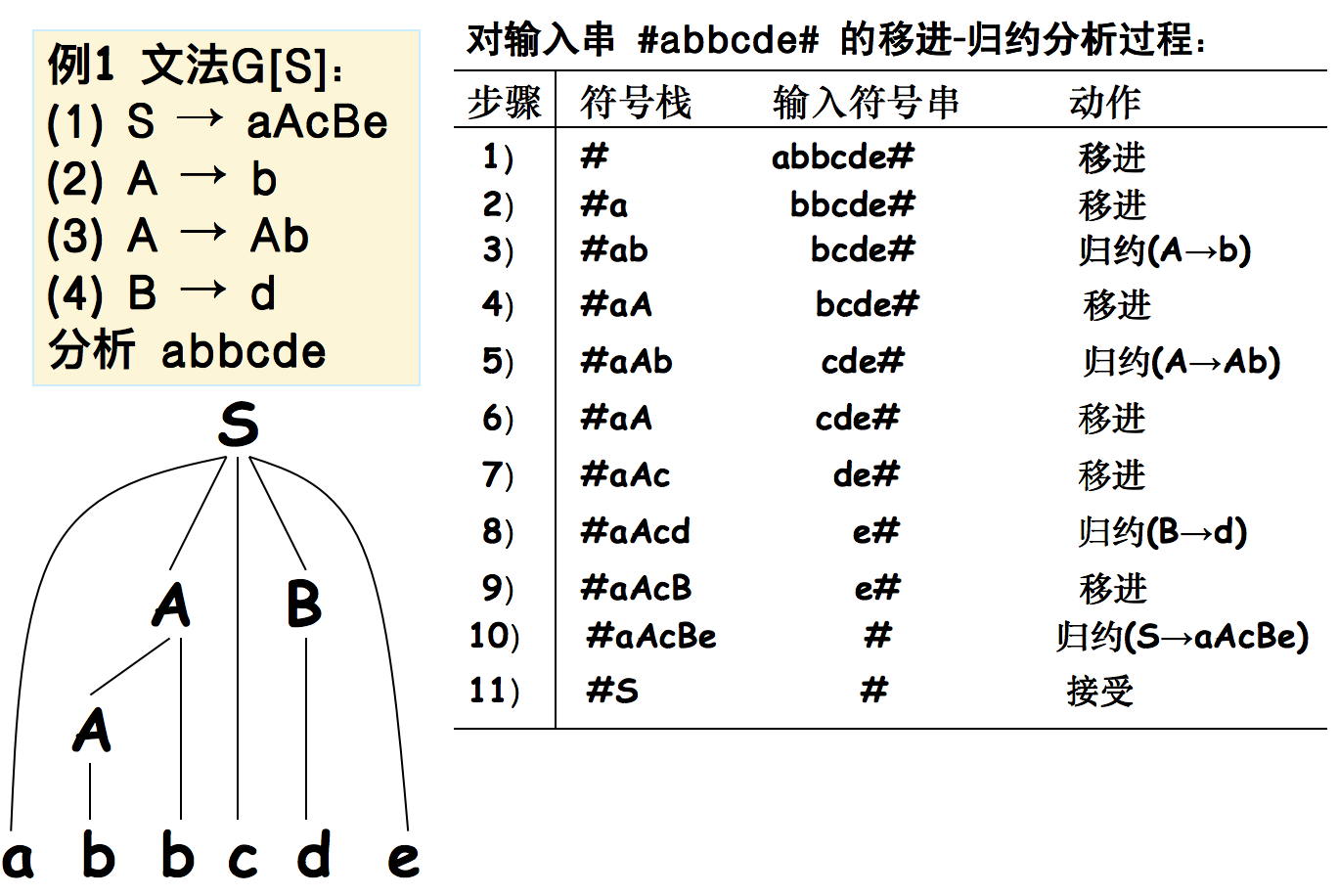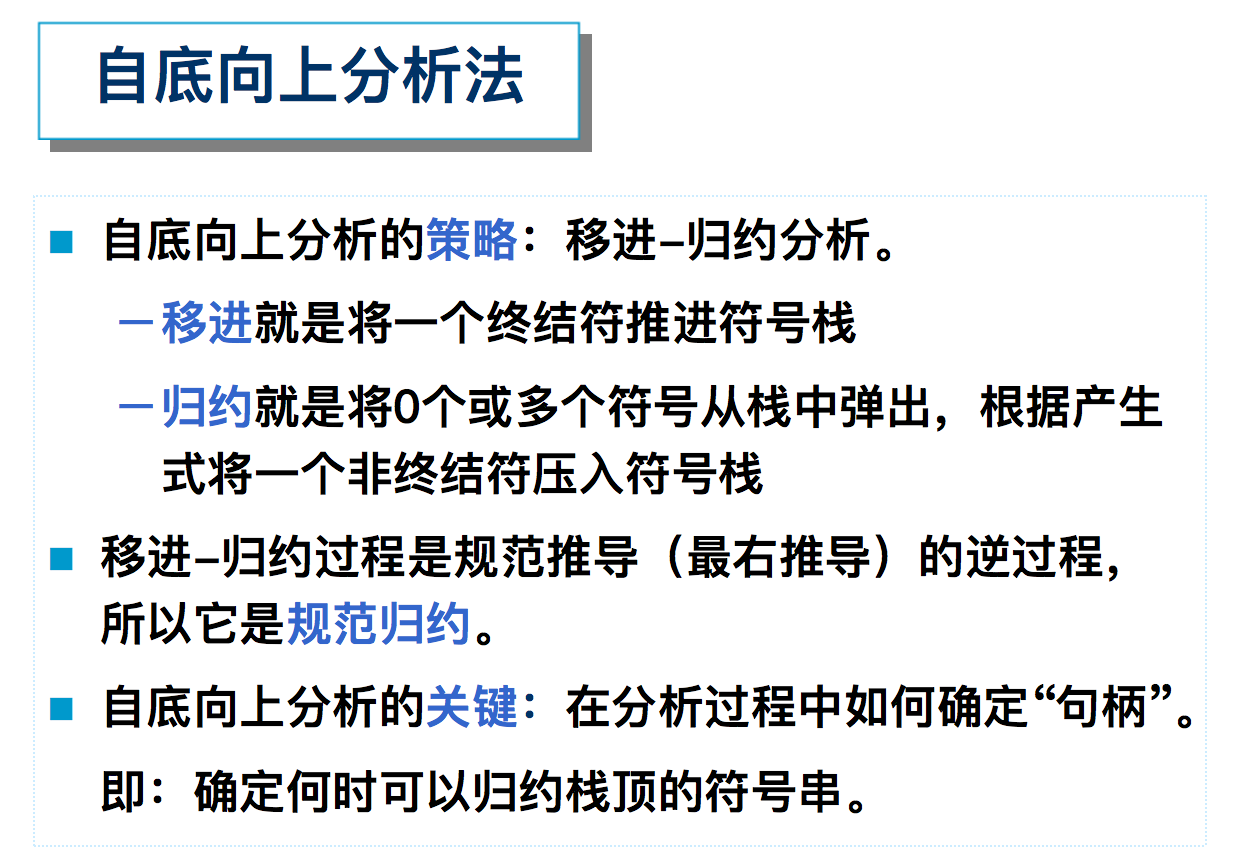5.1 自底向上优先分析法概述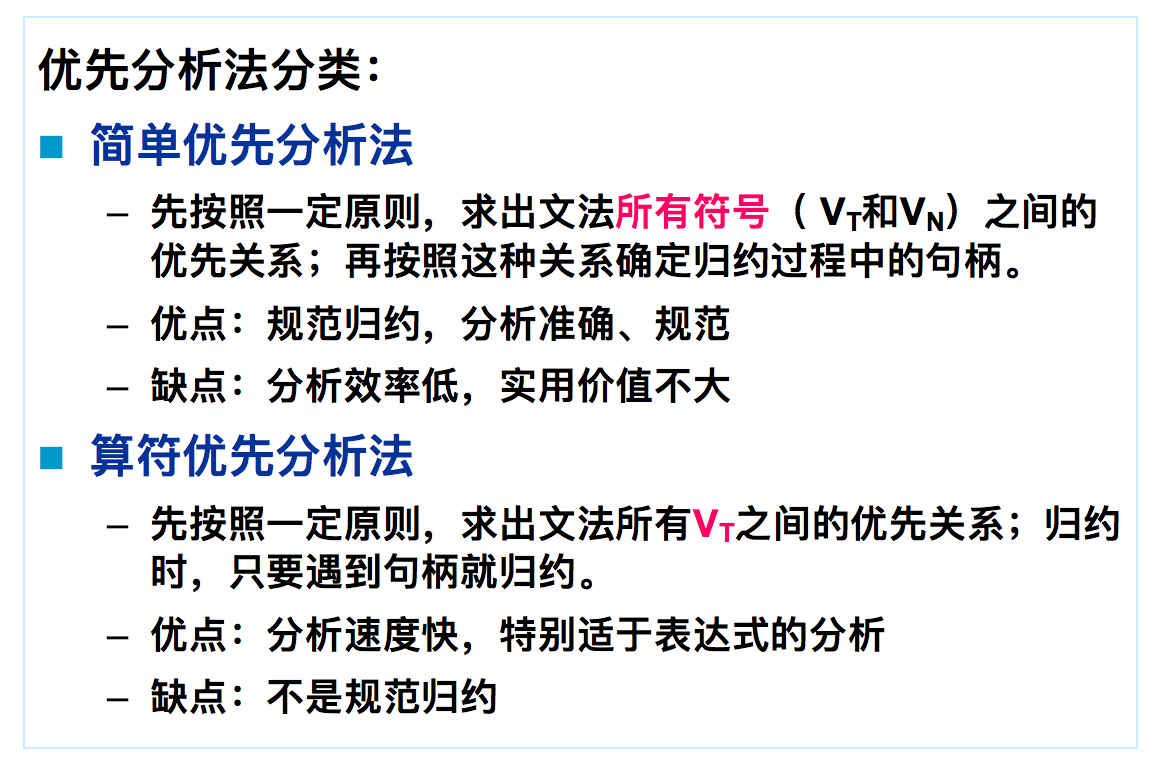5.2 简单优先分析法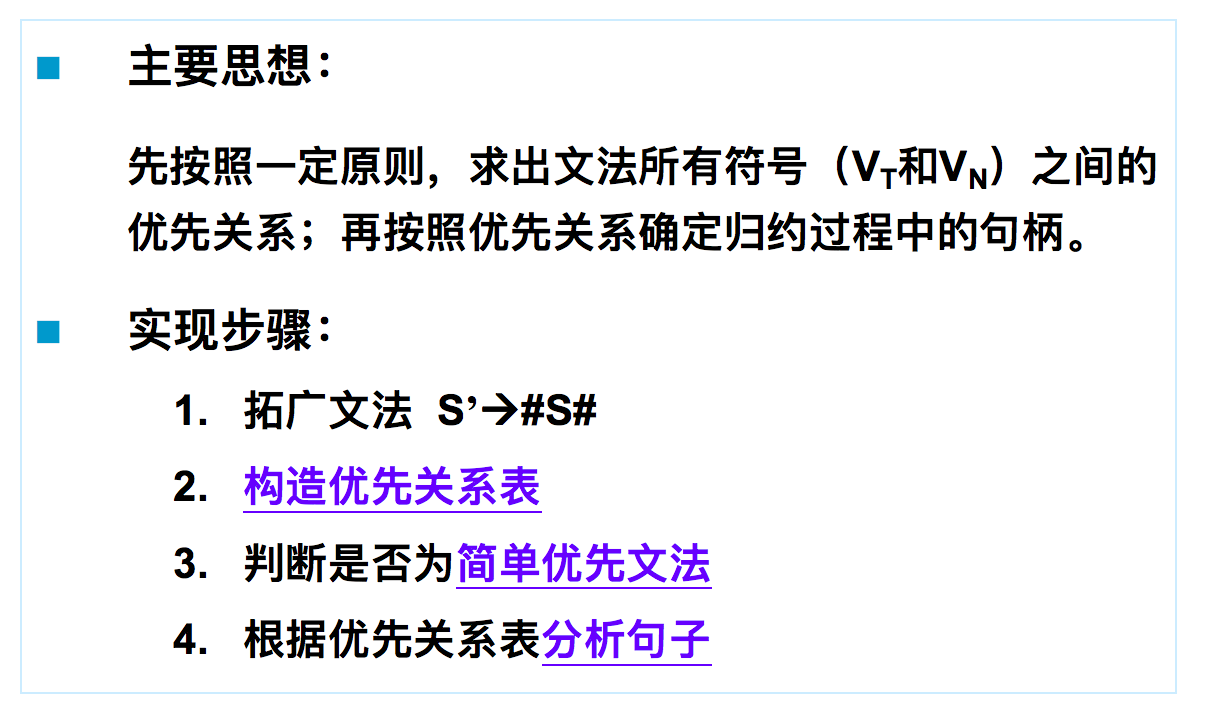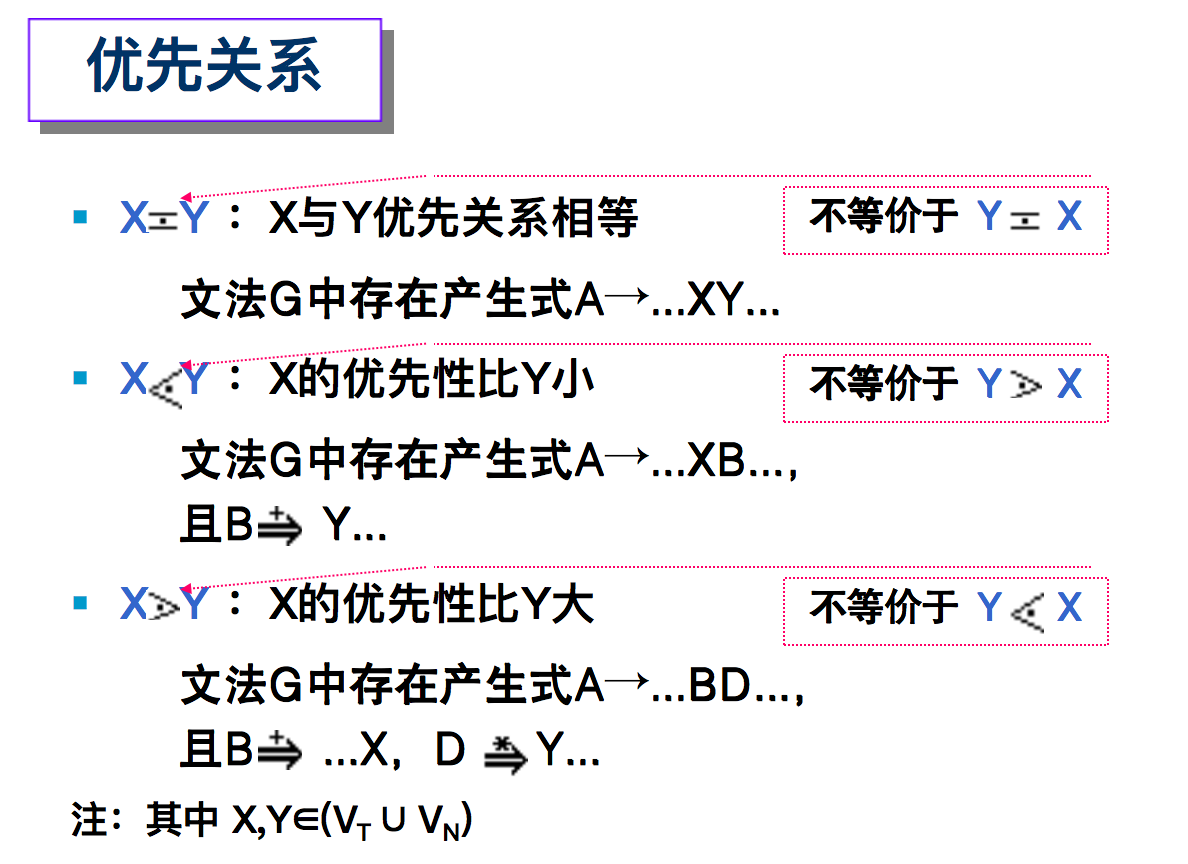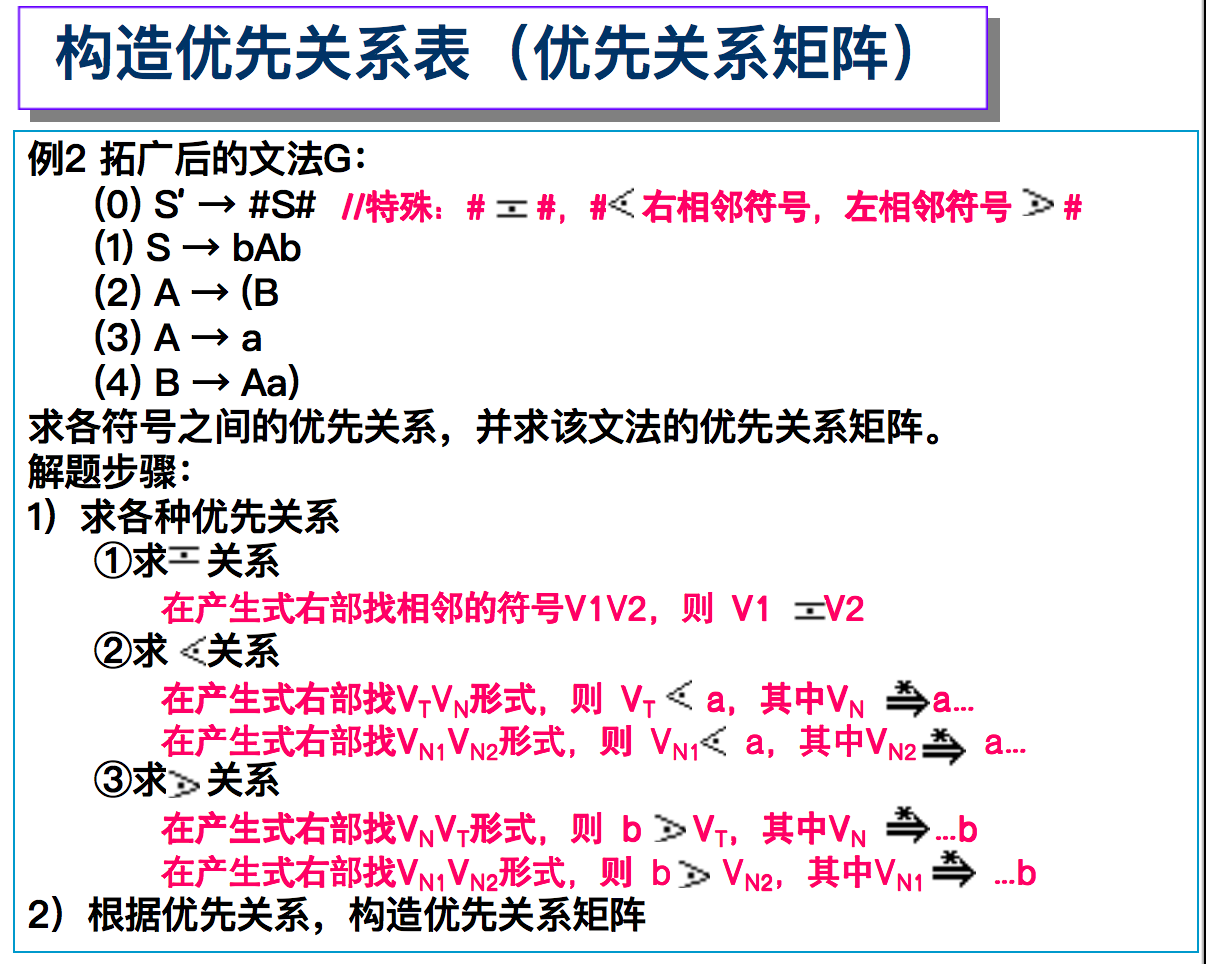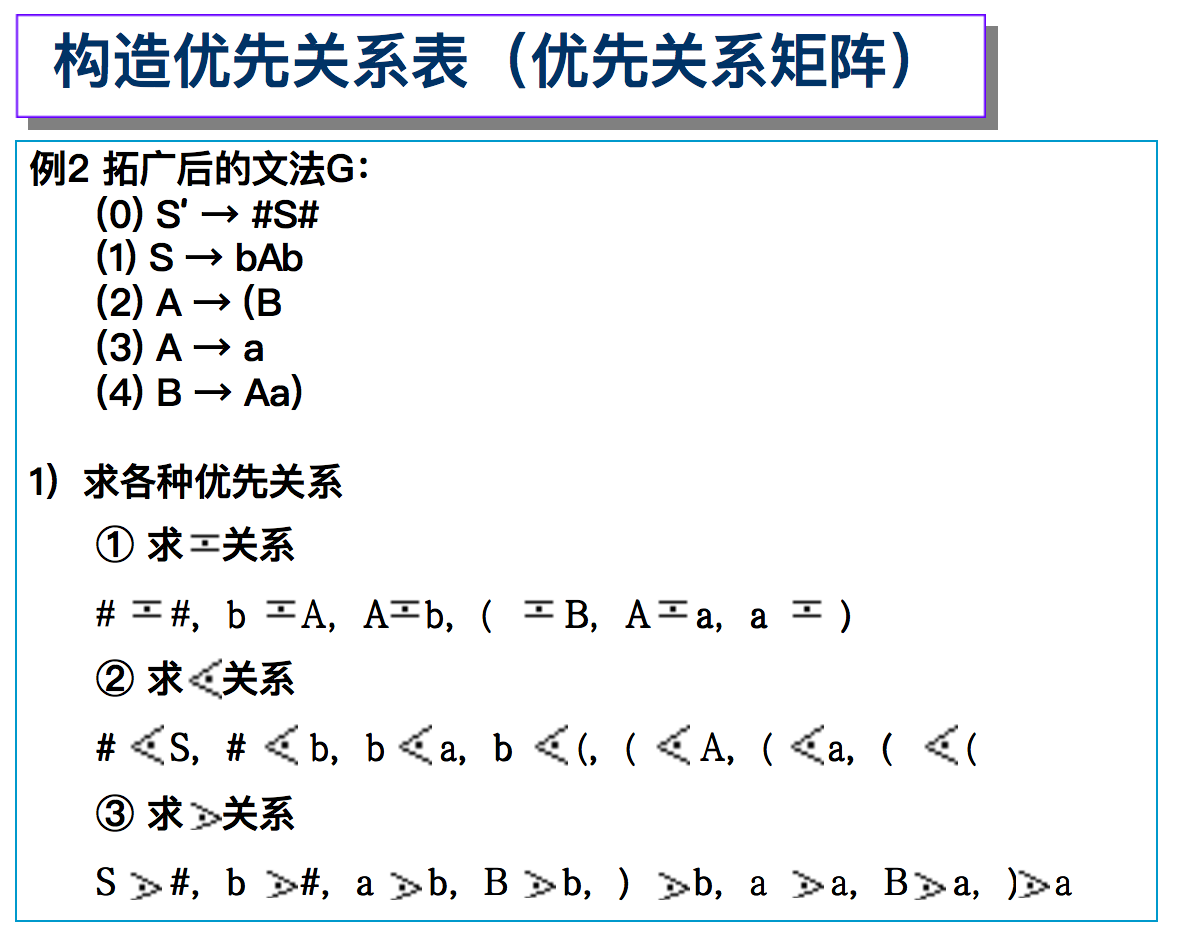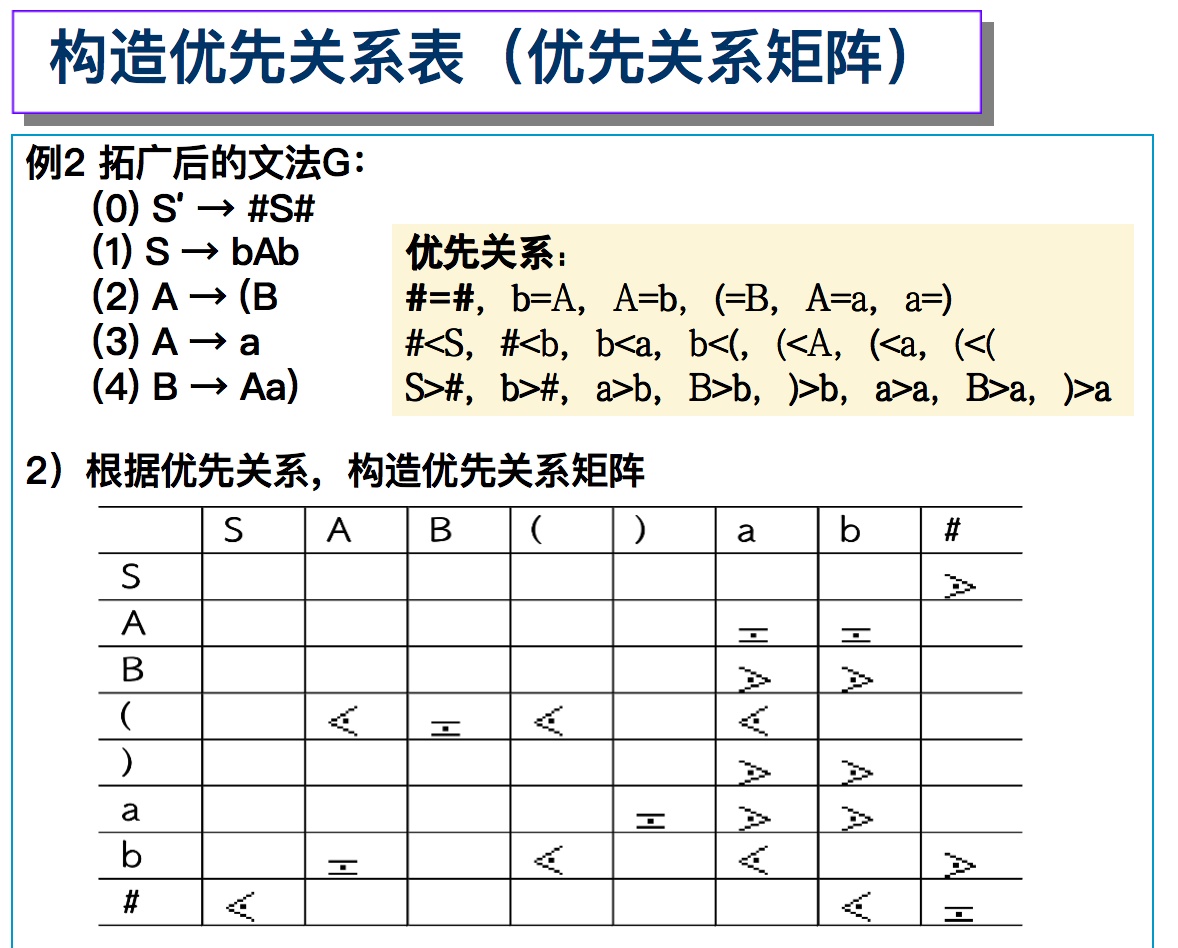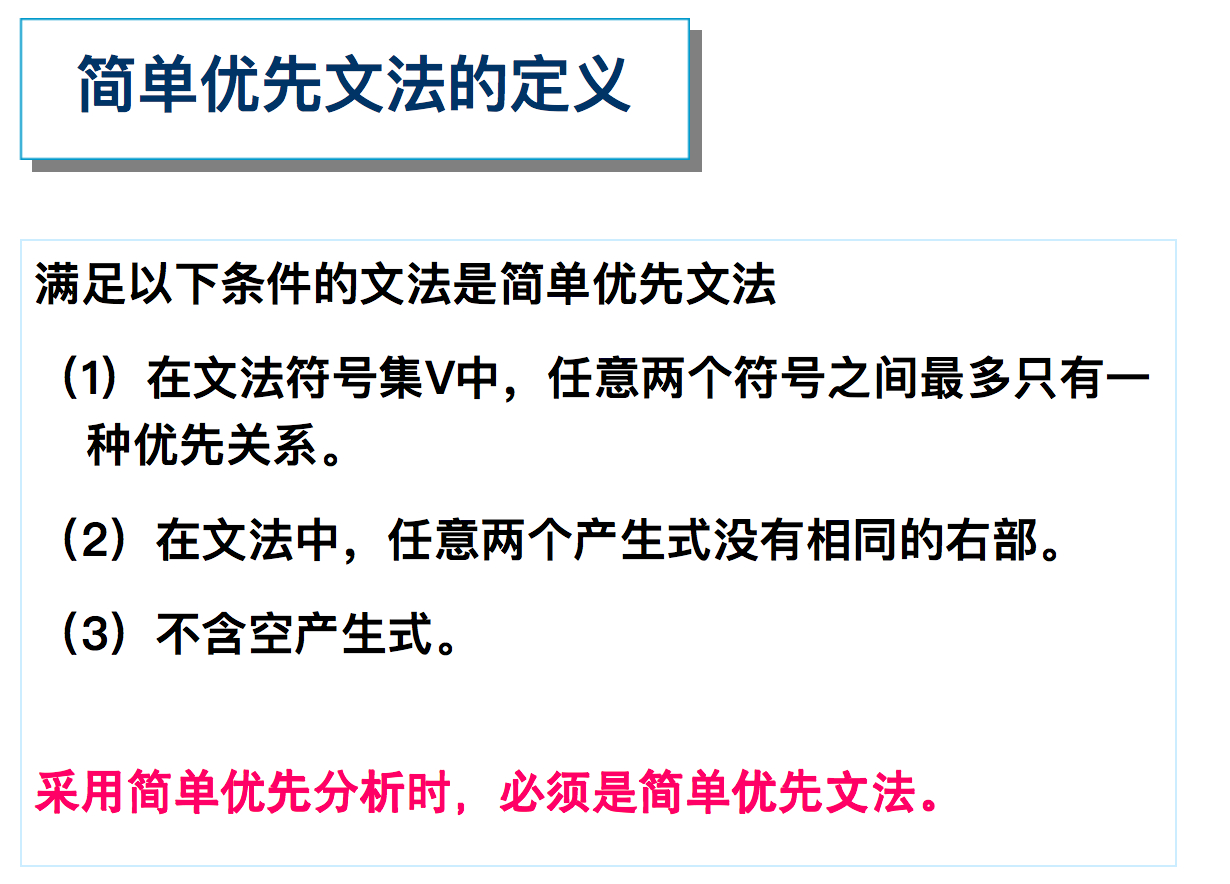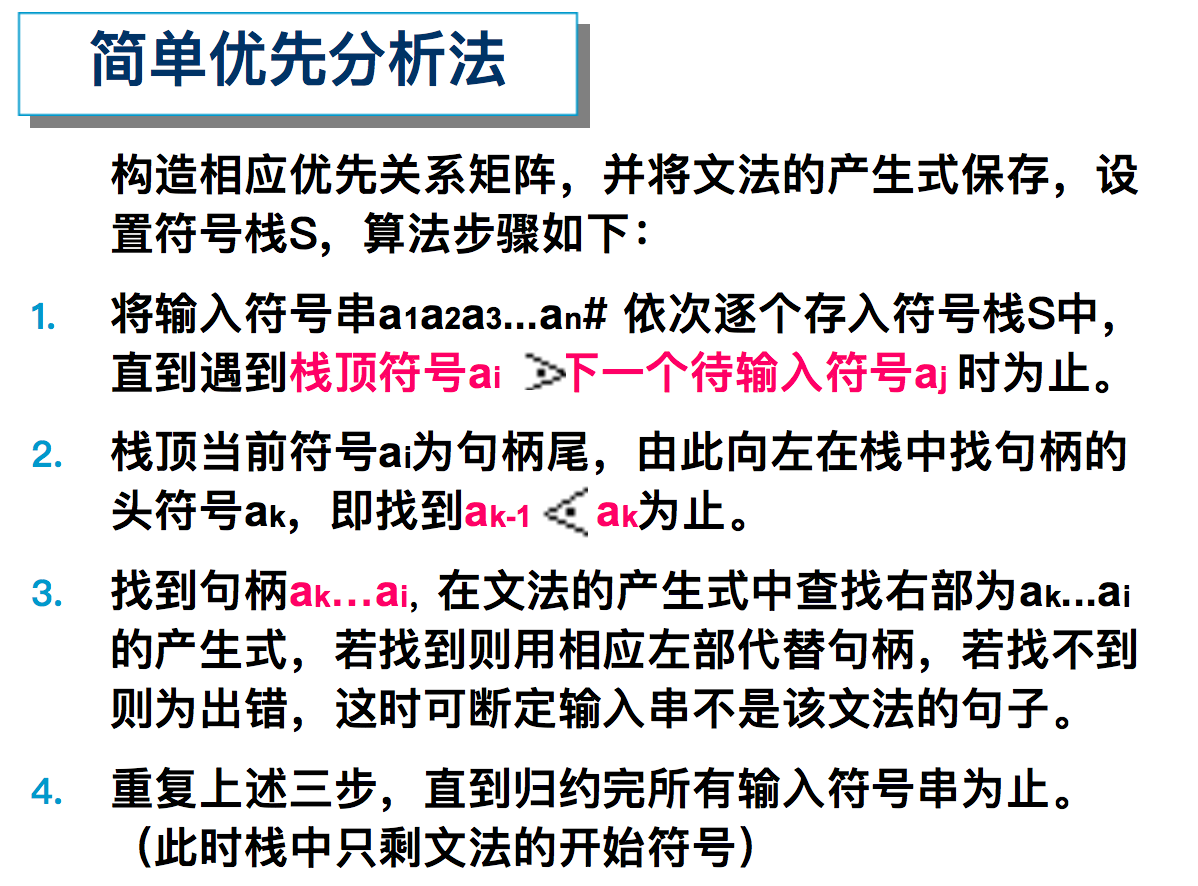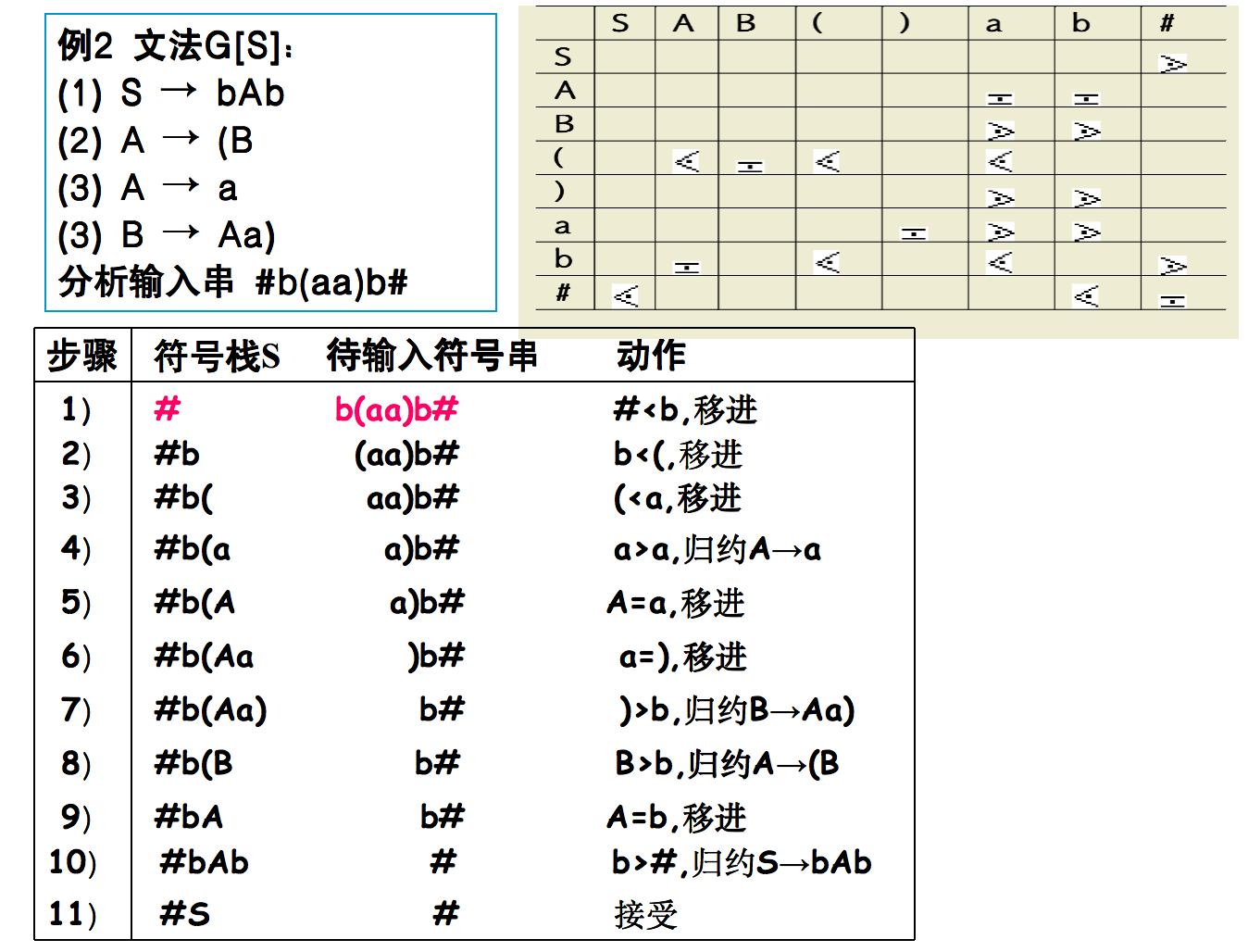5.3 算符优先分析法